# JP4182444B2 - Signal processing apparatus, signal processing method, and program - Google Patents

## Info

Publication number
JP4182444B2
JP4182444B2 JP2006160578A JP2006160578A JP4182444B2 JP 4182444 B2 JP4182444 B2 JP 4182444B2 JP 2006160578 A JP2006160578 A JP 2006160578A JP 2006160578 A JP2006160578 A JP 2006160578A JP 4182444 B2 JP4182444 B2 JP 4182444B2
Authority
JP
Japan
Prior art keywords
noise
input signal
max
signal
frame
Prior art date
Legal status (The legal status is an assumption and is not a legal conclusion. Google has not performed a legal analysis and makes no representation as to the accuracy of the status listed.)
Expired - Fee Related
Application number
JP2006160578A
Other languages
Japanese (ja)
Other versions
JP2007328228A (en
Inventor

Original Assignee
ソニー株式会社
Priority date (The priority date is an assumption and is not a legal conclusion. Google has not performed a legal analysis and makes no representation as to the accuracy of the date listed.)
Filing date
Publication date
Application filed by ソニー株式会社 filed Critical ソニー株式会社
Priority to JP2006160578A priority Critical patent/JP4182444B2/en
Publication of JP2007328228A publication Critical patent/JP2007328228A/en
Application granted granted Critical
Publication of JP4182444B2 publication Critical patent/JP4182444B2/en
Application status is Expired - Fee Related legal-status Critical
Anticipated expiration legal-status Critical

• 230000000875 corresponding Effects 0 abstract 1

## Images

•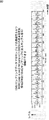•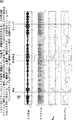•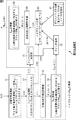•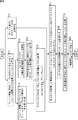•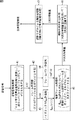•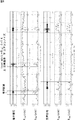•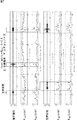•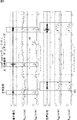•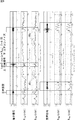•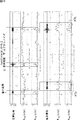•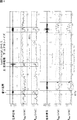•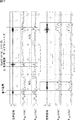•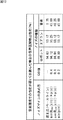•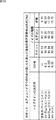•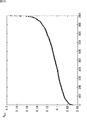•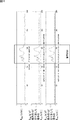•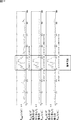•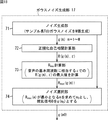•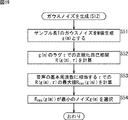•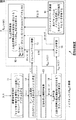•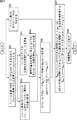••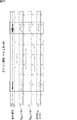•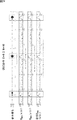•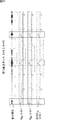•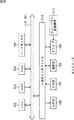## Classifications

• GPHYSICS
• G10MUSICAL INSTRUMENTS; ACOUSTICS
• G10LSPEECH ANALYSIS OR SYNTHESIS; SPEECH RECOGNITION; SPEECH OR VOICE PROCESSING; SPEECH OR AUDIO CODING OR DECODING
• G10L25/00Speech or voice analysis techniques not restricted to a single one of groups G10L15/00-G10L21/00
• G10L25/78Detection of presence or absence of voice signals
• GPHYSICS
• G10MUSICAL INSTRUMENTS; ACOUSTICS
• G10LSPEECH ANALYSIS OR SYNTHESIS; SPEECH RECOGNITION; SPEECH OR VOICE PROCESSING; SPEECH OR AUDIO CODING OR DECODING
• G10L25/00Speech or voice analysis techniques not restricted to a single one of groups G10L15/00-G10L21/00
• G10L25/90Pitch determination of speech signals

## Abstract

A signal processing device for processing an input signal includes gain calculating means and feature quantity calculating means. The gain calculating means is configured to obtain information indicating magnitude of noise to be added to the input signal on a basis of periodicity information indicating periodicity of the input signal and power of the input signal. The feature quantity calculating means is configured to obtain periodicity information of a noise-added signal obtained by adding noise having magnitude corresponding to the gain information to the input signal as a feature quantity of the input signal.

## Description

The present invention relates to a signal processing device, a signal processing method, and a program, and in particular, for example, it is possible to obtain a feature amount such as autocorrelation or YIN that can accurately detect a period with a periodicity of an input signal. The present invention relates to a signal processing device, a signal processing method, and a program.

As periodicity information representing the periodicity of an audio signal, for example, there is autocorrelation. Autocorrelation is used as a feature amount for capturing voiced sound in speech recognition, speech section detection, and the like (see, for example, Patent Document 1 and Non-Patent Documents 1 to 6). In addition, the autocorrelation of the audio signal is also used, for example, for detecting the fundamental frequency (pitch frequency) of the audio (for example, see Non-Patent Document 7).

In addition to autocorrelation, for example, there is YIN recently proposed as periodicity information (see, for example, Non-Patent Document 8). YIN is used to detect the fundamental frequency of speech.

Here, the value of the autocorrelation becomes large when the periodicity is high, and becomes 0 when there is no periodicity. On the other hand, YIN has a value of 0 when the periodicity is high and a large value (1) when there is no periodicity, contrary to autocorrelation. Hereinafter, the case where autocorrelation is employed as the periodicity information will be described, but when adopting YIN as the periodicity information, 1-YIN is used instead of the normalized autocorrelation described later, or The maximum value of normalized autocorrelation may be read as the minimum value of YIN, and the minimum value of normalized autocorrelation may be read as the maximum value of YIN.

There are several types of autocorrelation calculation methods, one of which will be described below.

Now, a sample value at time t of a time-series input signal sampled at a predetermined sampling frequency is represented as X (t). A range of T samples from a certain time T, that is, from a certain time t to a time t + T−1 is called a frame, and the T of the nth frame (nth frame) from the head of the input signal. A time series of the sample values is represented as a frame (or frame data) x (n).

The autocorrelation R ′ (x (n), τ) of the frame x (n) of the input signal X (t) can be calculated by, for example, Expression (1).

... (1)

Here, the autocorrelation of a signal is a value representing the correlation between the signal and a signal obtained by shifting the same signal as the signal by the time τ, and the time τ is called a lag.

Further, the autocorrelation R ′ (x (n), τ) of the frame x (n) is expressed by T sample values X (t), X (t + 1),. The T average value may be subtracted from (t + T-1) and may be obtained using a subtraction result obtained by subtracting the T average value from 0.

The autocorrelation obtained by normalizing the autocorrelation R ′ (x (n), τ) obtained by the equation (1) is called a normalized autocorrelation.

Now, if the autocorrelation obtained by normalizing the autocorrelation R ′ (x (n), τ) obtained by the equation (1) is expressed as R (x (n), τ), the normalized autocorrelation R ( x (n), τ) is, for example, normalized autocorrelation R ′ (x (n), τ) in equation (1) with autocorrelation R ′ (x (n), 0) with lag τ = 0 That is, it is obtained by calculating the formula R (x (n), τ) = R ′ (x (n), τ) / R ′ (x (n), 0).

The maximum value of the normalized autocorrelation R (x (n), τ) when the lag τ is changed is that the input signal X (t) has complete periodicity, that is, a certain period. It is 1 if it is a time series of T 0 and its period T 0 is equal to or less than the time length (frame length) T of the frame.

Also, the normalized autocorrelation R (x (n), τ) is close to 0 when the magnitude of the lag τ is sufficiently larger than 0 unless the input signal X (t) has periodicity. Become. Note that the normalized autocorrelation R (x (n), τ) is 1 when the lag τ is 0.

From the above, the normalized autocorrelation R (x (n), τ) can take values from −1 to +1.

By the way, human voiced sound has high periodicity if not perfect.

That is, FIG. 1 is a waveform diagram of an audio signal of a human voiced sound. In FIG. 1, the horizontal axis represents time, and the vertical axis represents the amplitude (level) of the audio signal.

It can be seen from FIG. 1 that the voice signal of a human voiced sound has periodicity. The audio signal in FIG. 1 is sampled at a sampling frequency of 16 kHz. The basic frequency of the audio signal in FIG. 1 is about 260 Hz (about 60 samples (≈16 kHz / 260 Hz)).

The period (reciprocal number) of a human voiced sound is called a fundamental frequency (pitch frequency), and it is generally known that the fundamental frequency exists in a range of approximately 60 Hz to 400 Hz.

Now, let us say that the range where the fundamental frequency of human voiced sound exists is the fundamental frequency range, and adopt the human voice signal (human voice signal) as the input signal X (t). Autocorrelation R (x (n), τ) is calculated, the maximum value R max (x (x (n), τ) of normalized autocorrelation R (x (n), τ) in the range of lag τ corresponding to the fundamental frequency range n)) is a value close to 1 in a section of a voiced sound signal having periodicity.

Here, if the sampling frequency of the input signal X (t) is, for example, 16 kHz, and the basic frequency range is, for example, the range from 60 Hz to 400 Hz described above, 60 Hz is approximately 266 samples (= 16 kHz / 60 Hz). 400Hz corresponds to 40 samples (= 16kHz / 400Hz).

Accordingly, since the range of lag τ corresponding to the fundamental frequency range is sufficiently larger than 0, the maximum value R max (of normalized autocorrelation R (x (n), τ) in the range of lag τ corresponding to the fundamental frequency range ( x (n)) is a value close to 0 in a section having no periodicity.

As described above, the maximum value R max (x (n)) of the normalized autocorrelation R (x (n), τ) in the range of lag τ corresponding to the fundamental frequency range is theoretically periodic. Since the value becomes significant between a certain section and a certain section, it can be used as a feature value of the speech signal as the input signal X (t) for speech processing such as speech section detection and speech recognition.

Here, FIG. 2 shows an audio signal as the input signal X (t) and various signals (information) obtained by processing the audio signal.

The first from the top in FIG. 2 is a waveform diagram of an audio signal as the input signal X (t). 2, the horizontal axis represents time (sample point), and the vertical axis represents amplitude.

Note that the first audio signal X (t) from the top of FIG. 2 is sampled at a sampling frequency of 16 kHz.

The second from the top in FIG. 2 shows a frequency spectrum obtained by performing FFT (Fast Fourier Transform) on the first audio signal X (t). In the second from the top in FIG. 2, the horizontal axis represents time (frame), and the vertical axis represents a number for identifying a so-called bin (frequency component) of the FFT.

As the FFT, since 512 points (samples) were performed, one bin corresponds to approximately 32 Hz. Also, in the second from the top in FIG. 2, the magnitude of each frequency component is represented by shading.

The third from the top in FIG. 2 shows the normalized autocorrelation R (x (x (n)) in the range of the lag τ corresponding to the fundamental frequency range of the first audio signal X (t) (the frame x (n) obtained from the same). The maximum value R max (x (n)) of n), τ) is shown. In the third from the top in FIG. 2, the horizontal axis represents time (frame), and the vertical axis represents the maximum value R max (x (n)).

Here, the maximum value R max (x (n)) of the normalized autocorrelation R (x (n), τ) in the lag τ range corresponding to the fundamental frequency range is referred to as lag range maximum correlation R It is called max (x (n)).

The fourth from the top in FIG. 2 is the power of the first audio signal X (t) (the frame x (n) obtained from), that is, the sum of the squares of the T sample values of the frame x (n). A value obtained by taking log (logarithm) (hereinafter referred to as frame log power as appropriate) is shown. In the fourth from the top in FIG. 2, the horizontal axis represents time (frame), and the vertical axis represents frame log power.

In FIG. 2, the part enclosed by the rectangle represents the audio | voice area. That is, in FIG. 2, the portions surrounded by the first, second, and third rectangles from the left represent sections where “stop”, “emergency stop”, and “freeze” are spoken, respectively.

In the first audio signal X (t), the second frequency spectrum, and the fourth frame log power from the top of FIG. 2, there is no significant difference between the audio section and the other sections. It can be seen that it is difficult to detect the speech section by using the signal X (t), the frequency spectrum, and the frame log power.

On the other hand, the third maximum lag range correlation R max (x (n)) from the top of FIG. 2 is close to 1 in the speech interval, and is relatively smaller than 1 in the other intervals, to 0. It is close.

Therefore, it can be seen that the lag range maximum correlation R max (x (n)) is an effective feature amount for detection of a speech section.

U.S. Patent No. 6,055,499 Using of voicing features in HMM-based speech Recognition, D.L.Thomson, Chengalvarayan, Lucent, 2002 Speech Communication Robust Speech Recognition in Noisy Environments: The 2001 IBM Spine Evaluation System, B. Kingsbury, G. Saon, L. Mangu, M. Padmanabhan and R. Sarikaya, IBM, ICASSP2002 Extraction Methods for Voicing Feature for Robust Speech Recognition, Andras Zolnay, Ralf Schluter, and Hermann Ney, RWTH Aachen, EUROSPEECH2003 USING SPEECH / NON-SPEECH DETECTION TO BIAS RECOGNITION SEARCH ON NOISY DATA, Francoise Beaufays, Daniel Boies, Mitch Weintraub, Qifeng Zhu, Nuance Communications, ICASSP2003 VOICING FEATURE INTEGRATION IN SRI'S DECIPHER LVCSR SYSTEM, Martin Graciarena, Horacio Franco, Jing Zheng, Dimitra Vergyri, Andreas Stolcke, SRI, ICASSP2004 A LINKED-HMM MODEL FOR ROBUST VOICING AND SPEECH DETECTION, Sumit Basu, Microsoft Research, ICASSP2003 Analysis, enhancement and evaluation of five pitch determination techniques, Peter Vepre, Michael S. Scordilis, Pansonic, Univ. Miami, Speech Communication 37 (2002), pp249-270 YIN, a fundamental frequency estimator for speech and music, Alain de Cheveigne´, Hideki Kawahara, Japan Acoustic Society Am. 111 (4), April 2002

By the way, the maximum lag range correlation R max (x (n)) of the input signal X (t) is set to 1 even for sounds other than human voiced sounds, for example, sounds having periodicity (periodic noise). It may be close.

Therefore, depending on the maximum lag range correlation R max (x (n)) of the input signal X (t), it may be difficult to distinguish the periodic noise portion and the voiced sound portion in the input signal X (t). is there.

Therefore, Non-Patent Document 6 describes a method of detecting a speech section by adding Gaussian noise to an input signal and using the lag range maximum correlation of the noise-added signal that is the input signal to which the Gaussian noise is added. Yes.

In other words, since the maximum correlation of the lag range of Gaussian noise is close to 0, even if the input signal contains periodic noise, the result is obtained by adding Gaussian noise with a level sufficiently larger than the periodic noise to the input signal. Of the obtained noise-added signal, the maximum lag range correlation of only the periodic noise becomes a value close to 0 due to the influence of Gaussian noise.

Therefore, ideally, a noise-added signal that is an input signal to which Gaussian noise is added by adding Gaussian noise having a large level to the part of the input signal that contains only periodic noise (the part where no sound exists). Is a value close to 0 in a portion where no speech is present (portion containing only periodic noise), and a maximum lag range correlation that is close to 1 in a portion where speech is present.

By the way, if a large level of Gaussian noise is added not only to the part where the voice does not exist, but also to the part where the voice exists, the maximum lag range correlation of the noise-added signal with the Gaussian noise added is the voice. In a portion where voice is present as well as a portion where no voice is present, the value is close to 0, and it becomes difficult to distinguish a periodic noise portion from a voice portion (speech interval).

Therefore, when obtaining the maximum lag range correlation of a noise-added signal with Gaussian noise added to the input signal and using this maximum lag range correlation to detect a voice interval, the level of the Gaussian noise added to the input signal Therefore, it is important that the level of Gaussian noise added to the part where the voice of the input signal does not exist is increased and the level of Gaussian noise added to the part where the voice of the input signal exists is reduced. It is.

Therefore, in Non-Patent Document 6, as a first-stage process, a feature amount using autocorrelation of an input signal is obtained, and a speech section and a section that is not so are roughly determined for the entire input signal based on the feature amount. Is determined, and the level of Gaussian noise added to the input signal is determined using the variance of the input signal in the section determined to be a non-speech section. The feature amount using the autocorrelation of the noise-added signal obtained by adding the Gaussian noise of the level determined in the first stage processing to the signal is obtained as the feature amount of the input signal, and the final speech section is based on the feature amount. And a method for determining a non-voice segment.

However, in the first stage processing, depending on the feature amount using the autocorrelation of the input signal, it may not be possible to accurately determine the speech section and the non-speech section for the entire input signal. is there.

Then, in the method described in Non-Patent Document 6, when the determination of the speech section and the non-speech section, which is performed based on the feature amount using the autocorrelation of the input signal in the first stage process, is incorrect, An inappropriate level is determined as the level of Gaussian noise to be added to the input signal, and as a result, the final speech is performed based on the feature amount using the autocorrelation of the noise added signal in the second stage processing. The determination of the section and the non-speech section is also inaccurate, and it becomes difficult to accurately detect the voice section, particularly a section having periodicity such as a voiced sound part.

The present invention has been made in view of such a situation, and makes it possible to obtain an autocorrelation that can accurately detect a section having a periodicity of an input signal.

The signal processing device according to one aspect of the present invention is a fixed time of a product of a maximum value within a lag corresponding to a specific frequency range of normalized autocorrelation of an input signal and the power of the input signal. The minimum value, median, or average value is added to the input signal, and the maximum value within a lag corresponding to a specific frequency range of autocorrelation is 0 or a value close to 0 . a gain calculation means for obtaining a gain information indicating the size, characteristics for determining the input signal, the periodicity information of the noise addition signal obtained by adding the magnitude of the noise in accordance with the gain information, a feature quantity of the input signal A quantity calculating means.

One aspect signal processing method of the present invention, or program, and a maximum value within the range of lug corresponding to a specific range of frequencies of the autocorrelation normalized input signal, the product of the power of the input signal A minimum value, median, or average value within a certain time is added to the input signal, and a maximum value within a lag corresponding to a specific frequency range of autocorrelation is 0 or a value close to 0 consisting determined as the gain information indicating the amount of noise, the input signal, the periodicity information of the noise addition signal obtained by adding the magnitude of the noise in accordance with the gain information, a step of determining a characteristic quantity of said input signal Including.

In one aspect of the present invention, a product of a maximum value within a lag corresponding to a specific frequency range of normalized autocorrelation of an input signal and the power of the input signal within a certain time. A noise value such that a minimum value, a median, or an average value is added to the input signal, and a maximum value within a lag range corresponding to a specific frequency range of autocorrelation is 0 or a value close to 0. obtained as gain information indicating, to the input signal, periodicity information for noise addition signal obtained by adding the magnitude of the noise in accordance with the gain information is obtained as the feature quantity of the input signal.

According to one aspect of the present invention, for example, periodicity information that can accurately detect an interval in which an input signal has periodicity can be obtained.

Embodiments of the present invention will be described below with reference to the drawings.

FIG. 3 is a block diagram showing a configuration example of an embodiment of a signal processing device to which the present invention is applied.

In the signal processing apparatus of FIG. 3, gain information indicating the magnitude of noise added to the input signal is obtained from the input signal, and noise having a magnitude (level) corresponding to the gain information is added to the input signal. The autocorrelation of the additional signal is obtained as a feature quantity of the input signal.

That is, in FIG. 3, the signal processing apparatus includes an acoustic signal conversion unit 11, a frame processing unit 12, a normalized autocorrelation calculation unit 13, an R max calculation unit 14, a frame power calculation unit 15, a gain calculation unit 16, and a Gaussian noise generation. A unit 17, a noise mix unit 18, a normalized autocorrelation calculation unit 19, and an R max calculation unit 20 are configured.

The acoustic signal conversion unit 11 includes, for example, a microphone (microphone) and an A / D (Analog / Digital) converter, and converts audio into a digital audio signal and supplies the digital audio signal to the frame processing unit 12.

That is, the acoustic signal conversion unit 11 converts sound (air sound or sound existing in the environment where the signal processing device is installed) as air vibrations input thereto into an analog sound signal using a microphone. . Further, the acoustic signal conversion unit 11 converts an analog audio signal obtained by the microphone into a digital audio signal by an A / D converter, and the audio signal is input to the frame processing unit 12 as a time series. To supply. Here, the sample value of the input signal at time t is represented as X (t).

The frame processing unit 12 performs frame processing for framing the input signal X (t) supplied from the acoustic signal conversion unit 11 into a frame composed of sample values of T samples, that is, for example, time t-T T sample values X (t−T + 1), X (t−T + 2),..., X (t) of the input signal from +1 to time t are one frame, and time t−T A sample value of T input signals from a time starting from a time after a predetermined frame shift time from +1 is set as one frame, and hereinafter, the input signal X ( A frame is formed from t) and supplied to the normalized autocorrelation calculation unit 13, the frame power calculation unit 15, and the noise mixing unit 18.

Here, hereinafter, the n-th frame from the top of the input signal (t) (the frame having the frame number n) will be referred to as a frame x (n) as appropriate.

The normalized autocorrelation calculation unit 13 obtains the autocorrelation R ′ (x (n), τ) of the frame x (n) supplied from the frame processing unit 12 according to, for example, the above equation (1), and further Then, the normalized autocorrelation R (x (n), τ) is obtained by normalizing the autocorrelation R ′ (x (n), τ).

Here, normalized autocorrelation R (x (n), τ) is also autocorrelation R ′ (x (n), τ) before being normalized to the normalized autocorrelation R (x (n), τ). ) Is also “autocorrelation”. The autocorrelation R ′ (x (n), τ) before normalization is hereinafter referred to as pre-normalization autocorrelation as appropriate.

As described above, the normalized autocorrelation R (x (n), τ) is the autocorrelation R ′ (x (n), τ) before normalization, the autocorrelation R ′ ( By normalizing with x (n), 0), that is, calculate the formula R (x (n), τ) = R '(x (n), τ) / R' (x (n), 0) Can be obtained.

When the normalized autocorrelation calculation unit 13 obtains the normalized autocorrelation R (x (n), τ) of the frame x (n), the normalized autocorrelation R (x (n), τ) is calculated as R max. It supplies to the calculation part 14.

R max calculation unit 14, for example, a range of frequencies from 80Hz to 400 Hz, a fundamental frequency range, the normalized autocorrelation R supplied from the normalized autocorrelation calculating unit 13 (x (n), τ ), A lag range maximum correlation R max (x (n)), which is the maximum value of the normalized autocorrelation R (x (n), τ) in the lag τ range corresponding to the fundamental frequency range, is obtained, and the gain calculation unit 16 To supply.

Here, as described above, when the basic frequency range is a frequency range from 80 Hz to 400 Hz, if the sampling frequency of the input signal X (t) in the acoustic signal converter 11 is, for example, 16 kHz, the basic frequency range is The range of lag τ corresponding to the frequency range is from 40 samples (= 16 kHz / 400 Hz) to 200 samples (= 16 kHz / 80 Hz). In this case, the Rmax calculator 14 obtains the maximum normalized autocorrelation R (x (n), τ) in the range where the lag τ is 40 to 200, and the lag range maximum correlation R max (x (n)) And

The frame power calculation unit 15 obtains power (hereinafter referred to as frame power) p (n) of the frame x (n) supplied from the frame processing unit 12 and supplies it to the gain calculation unit 16.

Here, the frame power calculation unit 15 calculates, for example, the sum of squares of each of the T sample values of the frame x (n) and the square root of the sum, and the calculation result is calculated as the frame power p (n). To do.

The gain calculator 16 supplies the lag range maximum correlation R max (x (n)) of the frame x (n) as the autocorrelation of the input signal X (t) supplied from the R max calculator 14 and the frame power. Based on the frame power p (n) of the frame x (n) supplied from the calculation unit 15 as the power of the input signal X (t), each frame x (n) ( Gain gain (n), which is gain information indicating the magnitude of noise added to the sample value), is obtained and supplied to the noise mixing unit 18.

That is, for example, the gain calculation unit 16 calculates the lag range maximum correlation R max (x (n)) of the frame x (n) from the R max calculation unit 14 and the frame x (n) from the frame power calculation unit 15. A predetermined function F (p (n), R max (x (n))) using the frame power p (n) as an argument is calculated, and the calculation result is used as a gain gain (n). To supply.

Here, as the function F (p (n), R max (x (n))) for obtaining the gain gain (n), for example, consecutive N frames including the frame x (n) (N is an integer of 2 or more) ), The minimum value of the product p (n) × R max (x (n)) of the frame power p (n) and the maximum lag range correlation R max (x (n)) (for each N frame) in the product p (n) × R max ( x (n)), the minimum value of the product p (n) × R max ( x (n))) may be employed such as a function for obtaining the.

The Gaussian noise generation unit 17 generates Gaussian noise of T samples equal to the number of samples in one frame as noise g to be added to the frame x (n) of the input signal X (t), and supplies the generated noise to the noise mixing unit 18. .

Note that the noise g generated in the Gaussian noise generation unit 17 is not limited to Gaussian noise, and may be any noise in which the lag range maximum correlation R max (g) of the noise g is 0 or a value close to 0. Any noise is acceptable.

The noise mixing unit 18 adds a noise having a magnitude corresponding to the gain gain (n) from the gain calculation unit 16 to the frame x (n) of the input signal X (t) from the frame processing unit 12. Is supplied to the normalized autocorrelation calculation unit 19.

That is, the noise mixing unit 18 converts the noise g from the Gaussian noise generation unit 17 into noise having a magnitude corresponding to the gain gain (n) from the gain calculation unit 16 (hereinafter referred to as level conversion noise as appropriate). Then, the frame y (n) of the noise added signal Y (t) obtained by adding the level conversion noise to the frame x (n) of the input signal X (t) from the frame processing unit 12 is obtained, and the normalized autocorrelation calculating unit 19 To supply.

Here, when the level conversion noise at time t is represented as B (t) and the noise addition signal at time t is represented as Y (t), the level conversion noise B (t) is added to the input signal X (t). The (added) signal X (t) + B (t) becomes the noise added signal Y (t).

Further, assuming that the nth frame (the time series of T sample values) from the head of the noise addition signal Y (t) is expressed as y (n), the noise mixing unit 18 sets C to a predetermined appropriate value. As a constant, for example, the frame y (n) of the noise added signal Y (t) is obtained according to the equation y (n) = x (n) + C × gain (n) × g.

The normalized autocorrelation calculation unit 19 performs the pre-normalization autocorrelation R ′ of the frame y (n) of the noise added signal Y (t) from the noise mixing unit 18 in the same manner as the normalized autocorrelation calculation unit 13 described above. (y (n), τ) is obtained, and the normalized autocorrelation R ′ (y (n), τ) is normalized to obtain the normalized autocorrelation R (y (n), τ). Obtained and supplied to the R max calculator 20.

In the same manner as the R max calculation unit 14 described above, the R max calculation unit 20 sets, for example, a frequency range from 80 Hz to 400 Hz as a fundamental frequency range, and a noise addition signal supplied from the normalized autocorrelation calculation unit 19 For normalized autocorrelation R (y (n), τ) of Y (t), it is the maximum value of normalized autocorrelation R (y (n), τ) in the range of lag τ corresponding to the fundamental frequency range The lag range maximum correlation R max (y (n)) is obtained and output as a feature quantity extracted from the frame x (n) of the input signal X (t).

3, the normalized autocorrelation calculation unit 13, the R max calculation unit 14, the frame power calculation unit 15, the gain calculation unit 16, the Gaussian noise generation unit 17, the noise mixing unit 18, and the normalized autocorrelation. The calculation unit 19 and the R max calculation unit 20 use the lag range maximum correlation R max (y (n)) of the noise added signal Y (t) as the feature amount of the frame x (n) from the frame x (n). The noise mix Rmax calculation unit for obtaining the above is configured. The process for obtaining the lag range maximum correlation R max (y (n)) of the noise addition signal Y (t) performed in the noise mix Rmax calculation unit is hereinafter referred to as noise mix Rmax calculation process as appropriate.

Here, as described above, the lag range maximum correlation R max (y (n)) of the noise added signal Y (t) obtained by adding Gaussian noise to the input signal X (t) is obtained, and the lag range maximum correlation R max (y (n)) is used to detect the voice interval, etc., the level of Gaussian noise added to the input signal X (t) is appropriately adjusted, that is, the input signal X (t) It is important to increase the level of Gaussian noise added to the part where no voice exists, and to reduce the level of Gaussian noise added to the part where the voice of the input signal X (t) exists.

On the other hand, in the signal processing device of FIG. 3, as described above, in the noise mixing unit 18, the noise addition signal Y (t) according to the equation y (n) = x (n) + C × gain (n) × g Frame y (n) is obtained. That is, the noise mixing unit 18 adds noise C × gain (n) × g having a magnitude proportional to the gain gain (n) to the frame of the input signal X (t), thereby adding the noise added signal Y (t ) Frame y (n).

Therefore, if the frame x (n) of the input signal X (t) is not a frame in the voice section, the gain gain (n) is increased and the frame x (n) of the input signal X (t) is a frame in the voice section. , The gain gain (n) needs to be reduced. In the gain calculation unit 16, the function F (p (n), R max (x (n))) for obtaining the gain gain (n) is A function that can obtain the gain gain (n) as described above is employed.

Here, as described in, for example, the document CONSTRUCTION AND EVALUATION OF A ROBUST MULTIFEATURE SPEECH / MUSICDISCRIMINATOR Eric Scheirer Malcolm Slaneyy ICASSP '97, pp. 1331-1334, for example, human (voice) In comparison, the ratio of frames whose frame power is smaller than the average value (average frame power) of the frame power in the section of about 1 second is high, that is, many frames have a frame power smaller than the average frame power. It is known that

Furthermore, as described in the above document, it is known that the spectrum of human speech changes at about 4 Hz (0.25 seconds).

From the above, for speech, it can be expected that power and normalized autocorrelation will change within a time of several hundred milliseconds (0. several seconds) to 1 second.

That is, it can be expected that the voice has a portion where the power greatly fluctuates and a portion where the autocorrelation greatly fluctuates in a time of several hundred milliseconds to one second. Therefore, for speech, it is a value calculated from power and autocorrelation, for example, product p (n) × R max of frame power p (n) and normalized autocorrelation R max (x (n)) It can be expected that (x (n)) fluctuates greatly in a time of several hundred milliseconds to about 1 second, and a small value exists.

On the other hand, for stationary noise such as music, it cannot be expected that there will be a portion where the power fluctuates greatly in the time of several hundred milliseconds to one second. Furthermore, the autocorrelation of stationary noise is uniformly large. Therefore, for stationary noise, for example, the product p (n) × R max (x (n)) of the frame power p (n) and the normalized autocorrelation R max (x (n)) described above is It cannot be expected that the time will vary greatly from several hundred milliseconds to about 1 second, and in particular, it is expected to become a relatively large value due to the effect of normalized autocorrelation R max (x (n)). be able to.

Therefore, as the function F (p (n), R max (x (n))) for obtaining the gain gain (n), for example, the frame power p (n) and the normalized autocorrelation R max (x (n)) P (n) × R max (x (n)) is the minimum value within a time of several hundred milliseconds to 1 second, so that the voice (frame x (n)) is It can be expected that a gain gain (n) having a small value is obtained, and that a gain gain (n) having a large value is obtained for stationary noise (frame x (n)).

Note that the function F () for obtaining the gain gain (n) is not limited to the above-described function. That is, the function F () for obtaining the gain gain (n) increases the maximum lag range correlation R max (y (n)) obtained for the frame of the speech section in the R max calculation unit 20, and increases the non-speech section. Any function that reduces the maximum lag range correlation R max (y (n)) required for a given frame may be used.

In the noise mixing unit 18, a constant used when obtaining the frame y (n) of the noise added signal Y (t) according to the equation y (n) = x (n) + C × gain (n) × g. As C, for example, the value of the constant C is changed to obtain the lag range maximum correlation R max (y (n)) of the noise added signal Y (t), and the lag range maximum correlation R max (y (n) ) Is used to conduct an experiment for detecting a speech segment, and the value of the constant C when the speech segment can be detected more accurately can be adopted.

Further, as the constant C used in the noise mixing unit 18, the value of the constant C is changed to obtain the lag range maximum correlation R max (y (n)) of the noise added signal Y (t), and the lag range. check visually by plotting the maximum correlation R max (y (n)), the value is large in the speech section, and obtained a lag range values in non-speech section is smaller maximum correlation R max (y (n)) The value of the constant C can be used.

Next, the operation of the signal processing apparatus of FIG. 3 will be described with reference to the flowchart of FIG.

In the signal processing apparatus of FIG. 3, an audio signal as an input signal X (t) is supplied from the acoustic signal conversion unit 11 to the frame processing unit 12.

In step S11, the frame processing unit 12 performs frame processing for framing the input signal X (t) supplied from the acoustic signal conversion unit 11 into a frame composed of sample values of T samples, and the result is obtained. The frame x (n) is supplied to the normalized autocorrelation calculation unit 13, the frame power calculation unit 15, and the noise mixing unit 18.

In step S <b> 13, the normalized autocorrelation calculation unit 13 obtains the normalized autocorrelation R (x (n), τ) of the frame x (n) from the frame processing unit 12 and supplies it to the R max calculation unit 14.

In step S14, the R max calculator 14 calculates the normalized self-correlation R (x (n), τ) from the normalized autocorrelation calculator 13 within the range of the lag τ corresponding to the fundamental frequency range. The maximum lag range correlation R max (x (n)), which is the maximum value of the correlation R (x (n), τ), is obtained and supplied to the gain calculation unit 16.

In step S15, the frame power calculation unit 15 obtains the frame power p (n) of the frame x (n) from the frame processing unit 12, and supplies the frame power p (n) to the gain calculation unit 16.

In step S <b> 16, the gain calculation unit 16 determines the lag range maximum correlation R max (x (n)) of the frame x (n) from the R max calculation unit 14 and the frame x (n) of the frame power calculation unit 15. Based on the frame power p (n), a gain gain (n) is obtained and supplied to the noise mixing unit 18.

That is, for example, the gain calculation unit 16 uses the frame power p (n) and the lag range maximum correlation R for N frames existing within a time of about several hundred milliseconds to 1 second around the frame x (n). max the minimum value of (x (n)) the product p (n) × R max of the (x (n)), determined as the gain gain (n), and supplies the noise-mixer 18.

On the other hand, the Gaussian noise generating unit 17 generates Gaussian noise g of T samples that is the same as the number of samples in one frame in Step S <b> 12 and supplies the generated Gaussian noise g to the noise mixing unit 18.

In step S17, the noise mixing unit 18 calculates the product of the constant C and the gain gain (n) from the gain calculation unit 16 according to the equation y (n) = x (n) + C × gain (n) × g. C × gain (n) is obtained, and noise C × gain (n) × g obtained by multiplying the Gaussian noise g from the Gaussian noise generation unit 17 by the product C × gain (n) is obtained. Further, in step S17, the noise mixing unit 18 adds the noise C × to the frame x (n) from the frame processing unit 12 according to the equation y (n) = x (n) + C × gain (n) × g. By adding gain (n) × g, the frame y (n) of the noise added signal Y (t) is obtained and supplied to the normalized autocorrelation calculation unit 19.

In step S18, the normalized autocorrelation calculation unit 19 obtains the normalized autocorrelation R (y (n), τ) of the frame y (n) of the noise added signal Y (t) from the noise mixing unit 18. This is supplied to the R max calculator 20.

In step S19, the R max calculator 20 calculates the normalized autocorrelation R (y (n), τ) from the normalized autocorrelation calculator 19 within the range of the lag τ corresponding to the fundamental frequency range. The maximum correlation R max (y (n)), which is the maximum value of the correlation R (y (n), τ), is obtained. Then, Rmax calculator 20, in step S20, and outputs the lag range maximum correlation R max (y (n)) , as the feature amount extracted from a frame x (n) of the input signal X (t).

Next, FIG. 5 shows a configuration example of an embodiment of a speech segment detection device to which the signal processing device of FIG. 3 is applied.

In the speech segment detection apparatus of FIG. 5, the lag range maximum correlation R max of the noise added signal Y (t) obtained by adding noise to the input signal X (t) as the feature quantity of the speech signal as the input signal X (t). Using (y (n)), the speech section of the input signal X (t) is detected.

That is, in the speech section detection device of FIG. 5, the acoustic signal conversion unit 41 converts the sound as air vibration input thereto into an analog speech signal, like the acoustic signal conversion unit 11 of FIG. 3. Further, the analog audio signal is converted into a digital audio signal and supplied to the frame processing unit 42 as an input signal X (t).

The frame processing unit 42 performs frame processing for framing the input signal X (t) from the acoustic signal conversion unit 41 into a frame composed of sample values of T samples, similarly to the frame processing unit 12 of FIG. The frame x (n) obtained as a result is supplied to the noise mix R max calculation unit 43 and the frame power calculation unit 44.

Noise Mix R max calculation unit 43, noise mixes R max calculator of FIG. 3, i.e., the normalized autocorrelation calculating unit 13, R max calculator 14, the frame power calculating unit 15, gain calculation unit 16, a Gaussian noise generator 17, the noise mix unit 18, the normalized autocorrelation calculation unit 19, and the R max calculation unit 20, and the frame x (n supplied from the frame processing unit 42 by performing the noise mix R max calculation process. ), The maximum lag range correlation R max (y (n)) of the noise added signal Y (t) is obtained and supplied to the multiple frame processing unit 45.

On the other hand, the frame power calculation unit 44 calculates the frame log power of the frame x (n) from the frame x (n) from the frame processing unit 42, and further normalizes the normalized log power of the frame log power. logp (n) is obtained and supplied to the multiple frame processing unit 45.

That is, the frame power calculation unit 44 obtains the frame log power FP (n) by taking the log of the sum of the squares of the T sample values of the frame x (n).

Further, the frame power calculation unit 44, for example, calculates the formula FPave (n) = ff × FPave (n−1) + (1-ff) × FP (n) using the forgetting factor ff, thereby calculating FPave (n n) is obtained as an average value of the frame log power FP (n).

Then, the frame power calculation unit 44 subtracts the average value FPave (n) from the frame log power FP (n), and the subtraction value FP (n) −FPave (n) is normalized log power logp (n). Is supplied to the multiple frame processing unit 45.

Here, the normalized log power is obtained by subtracting the average value FPave (n) from the frame log power FP (n) and obtaining the normalized log power logp (n). The average of logp (n) is almost zero. That is, the frame power calculation unit 44 normalizes the frame log power FP (n) so that the average is zero.

The multiple frame processing unit 45 collects the lag range maximum correlation R max (y (n)) from the noise mix R max calculation unit 43 and the normalized log power logp (n) from the frame power calculation unit 44 ( And the feature amount (integrated feature amount) of the target frame of the input signal X (t) is obtained.

That is, when the n-th frame x (n) from the head of the input signal X (t) is now referred to as the frame of interest, the multiple frame processing unit 45 lags the frame of interest and several frames before and after it. range maximum correlation R max (y (n)), and a vector of normalized log power logp a (n) and the component is obtained as the feature of the subject frame.

Specifically, a plurality frame processing unit 45, for example, the lag range maximum correlation R max of the frame of interest (y (n)) and its eight frames each before and after the lag range maximum correlation R max (y (n)) In total, 17 lag range maximum correlations R max (y (n)) are sorted in ascending order, and the normalized log power logp (n) of the frame of interest and the normalized log of each of the 8 frames before and after that A total of 17 normalized log powers logp (n) with power logp (n) are sorted in ascending order, and 17 lag range maximum correlations R max (y (n)) after sorting and after sorting A 34-dimensional vector having 17 normalized log powers logp (n) as components is obtained as a feature amount of the frame of interest.

Then, the multiple frame processing unit 45 supplies a 34-dimensional vector as the feature amount of the frame of interest to the linear discriminant analysis unit 46.

The linear discriminant analysis unit 46 compresses the dimension of the vector as the feature quantity of the frame x (n) from the multiple frame processing unit 45 and supplies it to the identification processing unit 47.

That is, the linear discriminant analysis unit 46 converts the 34-dimensional vector as the feature quantity of the frame x (n) from the multiple frame processing unit 45 into a two-dimensional vector by, for example, linear discriminant analysis (LDA (Linear Discriminant Analysis)). It is compressed into a vector and supplied to the identification processing unit 47 as the feature quantity of the frame x (n).

Based on the two-dimensional vector as the feature quantity from the linear discriminant analysis unit 46, the identification processing unit 47 identifies whether the frame x (n) is a frame of a speech section or a frame of a non-speech section. And the identification result is output as speech section information.

That is, the identification processing unit 47 stores, for example, an HMM (Hidden Markov Model) trained for detecting a speech section, and the feature quantity from the linear discriminant analysis unit 46 is observed in the HMM. Based on the degree, it is identified whether the frame x (n) is a frame of a speech section or a frame of a non-speech section, and the identification result is output as speech section information.

In Non-Patent Document 2, the lag range maximum correlation R max (y (n)) of the noise added signal Y (t) obtained by adding noise to the input signal X (t) is not the input signal X (t). Describes a method for detecting speech intervals using a five-state tied-state HMM with lag range maximum correlation R max (x (n)) and normalized log power logP (n) as features . The tied-state HMM here is a mixed Gaussian distribution (GMM: Gaussian Mixture) where each of the voice HMM and the non-voice HMM has five states, but the voice HMM and the non-voice HMM have the same five states. Model).

Detection of the speech segment to be performed by the speech segment detection device of FIG. 5, as the feature quantity, instead of the lag range maximum correlation R max of the input signal X (t) (x (n )), to the input signal X (t) in The point where the maximum lag range correlation R max (y (n)) of the noise-added signal Y (t) with noise added is used, and the tied-state HMM instead of the five-state tied-state HMM for voice segment identification This is different from the method described in Non-Patent Document 2 in that a normal five-state HMM is used.

Next, with reference to FIG. 6 to FIG. 14, the result of the speech segment detection experiment performed using the speech segment detection apparatus of FIG. 5 will be described.

In the experiment, the analog audio signal obtained by the microphone used in QRIO (R), a biped robot developed by Sony Corporation, was sampled at a sampling frequency of 16 kHz to produce a digital audio signal. Converted and used as input signal X (t).

Further, in the experiment, the frame length (number of samples) T was set to 1024 samples, and the frame x (n) was extracted from the input signal X (t) while shifting by 160 samples.

In the experiment, the average value FPave (n) used to obtain the normalized log power logp (n) is expressed by the formula FPave (n) = ff × FPave (n-1) + (1-ff) × FP (n ) Is used as the forgetting factor ff.

Furthermore, a mixed Gaussian distribution was adopted as the probability density function of the HMM used for speech segment identification. In addition to preparing an HMM for speech segments and an HMM for non-speech segments, an HMM learning input signal X (t) is prepared, and linear discrimination is performed from the learning input signal X (t). A two-dimensional vector similar to that obtained by the analysis unit 46 is obtained as a feature quantity, and the feature quantity obtained from the speech section of the learning input signal X (t) is given to the speech section HMM, Learning from the HMM for the non-speech section and the HMM for the non-speech section by giving the feature quantity obtained from the non-speech section of the learning input signal X (t) to the non-speech section HMM It was.

Further, in the experiment, a person labels the start and end frames of the speech section with respect to the experimental input signal X (t), and the speech section represented by the speech section information output from the identification processing unit 47 and the person Is compared with the voice section labeled with the start and end frames to determine whether the voice section represented by the voice section information output by the identification processing unit 47 is correct or not.

Specifically, the start and end frames of the speech section labeled by the person are the Ts frame and the Te frame, respectively, and the start of the speech section represented by the speech section information output by the identification processing unit 47 If the end frames are the Ss frame and the Se frame, respectively, Ss satisfies the formula Ts-40 <= Ss <= Ts, and Se satisfies the formula Te <= Se <= Te + 40. When it is satisfied, it is assumed that the voice section represented by the voice section information output by the identification processing unit 47 is correct.

In addition, in the experiment, as the function F (p (n), R max (x (n))) for obtaining the gain gain (n), the frame for each of the consecutive N frames including the frame x (n) A function for obtaining the minimum value of the product p (n) × R max (x (n)) of the power p (n) and the maximum lag range correlation R max (x (n)) (hereinafter referred to as the product minimum value function as appropriate) ), And the product of frame power p (n) and maximum lag range correlation R max (x (n)) for each of consecutive N frames including frame x (n) p (n) × R max The minimum value of the frame power p (n) for each of N consecutive frames including a function (hereinafter referred to as a product average value function) for obtaining an average value of (x (n)) and a frame x (n) as appropriate. A function to be obtained (hereinafter referred to as a power minimum value function as appropriate) was used.

Further, 40 frames were adopted as N frames for defining the function F (p (n), R max (x (n))).

FIG. 6 shows the lag range maximum correlation R max of the noise added signal Y (t) when the product minimum value function is adopted as the function F (p (n), R max (x (n))) in the experiment. (y (n)) is shown.

That is, the upper half side in FIG. 6 shows the lag range maximum correlation R max of the noise added signal Y (t) obtained as an input signal X (t), which is an audio signal collected in an environment (music environment) in which music is flowing. (y (n)) is shown, and in the lower half of FIG. 6, the audio signal collected in the environment (air conditioner environment) where the air conditioner (air conditioner) is operating is obtained as the input signal X (t). The lag range maximum correlation R max (y (n)) of the noise added signal Y (t) is shown.

The first from the upper half of FIG. 6 shows the audio signal collected in the music environment, that is, the input signal X (t), and the second from the top shows the lag of the input signal X (t). The range maximum correlation R max (x (n)) is shown. The third from the upper half of FIG. 6 shows the lag range maximum correlation R max (y (n)) of the noise added signal Y (t) obtained by adding noise to the input signal X (t).

The lower half of FIG. 6 also shows the audio signal collected in the air conditioner environment, that is, the input signal X (t), and the second from the top shows the first input signal X ( The maximum correlation R max (x (n)) of t) is shown. The third from the top in the upper half of FIG. 6 shows the lag range maximum correlation R max (y (n)) of the noise added signal Y (t) obtained by adding noise to the first input signal X (t). ing.

In FIG. 6, a portion surrounded by a vertically long rectangle represents a voice section. The same applies to FIG. 7 described later.

FIG. 7 shows the noise addition signal Y (t) when the product minimum value function is adopted as the function F (p (n), R max (x (n))) in the experiment, as in FIG. The lag range maximum correlation R max (y (n)) is shown.

However, in FIG. 6, 0.4 is adopted as the constant C that defines the equation y (n) = x (n) + C × gain (n) × g used to obtain the noise addition signal Y (t). On the other hand, in FIG. 7, 0.2 is adopted as the constant C. The other points of FIG. 7 are the same as those of FIG.

Compared to lag range maximum correlation R max of the input signal X of FIG. 6 and FIG. 7 (t) (x (n )), the lag range maximum correlation R max of the noise addition signal Y (t) and a (y (n)) Then, the lag range maximum correlation R max of the noise addition signal Y (t) (y (n )) , in the speech interval, the value of the lag range maximum correlation R max of the input signal X (t) (x (n )) In the non-voice section, the value is smaller than the lag range maximum correlation R max (x (n)) of the input signal X (t).

Therefore, the level of noise added to the input signal X (t) is appropriately adjusted in the gain calculation unit 16 in FIG. 3, and as a result, the sound of the input signal X (t) exists in the noise mixing unit 18. It can be seen that a large level of noise is added to the portion that is not, and a small level of noise is added to the portion where the sound of the input signal X (t) exists.

FIG. 8 shows the lag range maximum correlation R max of the noise-added signal Y (t) when the product average function is adopted as the function F (p (n), R max (x (n))) in the experiment. (y (n)) is shown.

That is, the upper half side of FIG. 8 shows the maximum correlation in the lag range of the noise added signal Y (t) obtained by using the audio signal collected in the music environment as the input signal X (t), as in the upper half of FIG. R max (y (n)) is shown, and the lower half of FIG. 8 shows the noise obtained as an input signal X (t), as in the case of the lower half of FIG. The lag range maximum correlation R max (y (n)) of the additional signal Y (t) is shown.

However, in FIG. 8, as described above, the product average value function is adopted as the function F (p (n), R max (x (n))) instead of the product minimum value function.

8 shows the audio signal collected in the music environment, that is, the input signal X (t), and the second from the top shows the lag of the input signal X (t). The range maximum correlation R max (x (n)) is shown. The third from the top in the upper half of FIG. 8 shows the lag range maximum correlation R max (y (n)) of the noise added signal Y (t) obtained by adding noise to the input signal X (t).

The lower half of FIG. 8 also shows the audio signal collected in the air conditioner environment, that is, the input signal X (t) from the top, and the second from the top shows the first input signal X ( The maximum correlation R max (x (n)) of t) is shown. The third from the top in the upper half of FIG. 8 shows the maximum lag range correlation R max (y (n)) of the noise added signal Y (t) obtained by adding noise to the first input signal X (t). ing.

In FIG. 8, a portion surrounded by a vertically long rectangle represents a voice section. The same applies to FIG. 9 described later.

FIG. 9 shows the noise addition signal Y (t) when the product average value function is adopted as the function F (p (n), R max (x (n))) in the experiment, as in FIG. The lag range maximum correlation R max (y (n)) is shown.

However, in FIG. 8, 0.1 is adopted as a constant C that defines the equation y (n) = x (n) + C × gain (n) × g used to obtain the noise addition signal Y (t). In contrast, in FIG. 9, 0.05 is adopted as the constant C. The other points of FIG. 9 are the same as those of FIG.

8, the portion indicated by A8 1, lag range maximum correlation R max of the noise addition signal Y (t) (y (n )) is, despite the non-speech section, the speech section and comparable values This indicates that a sufficiently large noise is not added to the input signal X (t).

Further, in FIG. 8, the portion indicated by A8 2, lag range maximum correlation R max of the noise addition signal Y (t) (y (n )) is, despite the speech section, the input signal X (t) in of which is smaller than the value of the lag range maximum correlation R max (x (n)) , which indicates that the level of added noise in the input signal X (t) in too large.

A larger constant C, the value of the non-speech interval of the lag range maximum correlation R max of the noise addition signal Y (t) (y (n )), i.e., for example, the value of the portion indicated by A8 1 in FIG. 8 Can be small. However, increasing the constant C, the value of the speech segment of the lag range maximum correlation R max of the noise addition signal Y (t) (y (n )), i.e., for example, the value of the portion indicated by A8 2 in FIG. 8 , Even smaller.

On the other hand, by decreasing the constant C, the value of the speech segment of the lag range maximum correlation R max of the noise addition signal Y (t) (y (n )), i.e., for example, a portion indicated by A8 2 in FIG. 8 by increasing the value, it can be set to a value about the same lag range maximum correlation R max of the input signal X (t) (x (n )).

However, when the constant C is reduced, as shown in FIG. 8 where the constant C is 0.1 and FIG. 9 where the constant C is less than 0.1, the maximum lag range correlation R max of the noise added signal Y (t) is understood. The value of (y (n)) in the non-speech interval cannot be reduced.

That is, when decreasing the constant C, and 9, as indicated by A9 1 and A9 2, lag range maximum correlation R max of the noise addition signal Y (t) (y (n )) is, in a non-speech section Regardless, the value is increased to the same level as that of the voice interval.

FIG. 10 shows the lag range maximum correlation R max of the noise added signal Y (t) when the power minimum value function is adopted as the function F (p (n), R max (x (n))) in the experiment. (y (n)) is shown.

That is, the upper half side of FIG. 10 shows the maximum correlation of the lag range of the noise added signal Y (t) obtained by using the audio signal collected in the music environment as the input signal X (t), as in the upper half of FIG. R max (y (n)) is shown, and the lower half of FIG. 10 shows the noise obtained as an input signal X (t), as in the case of the lower half of FIG. The lag range maximum correlation R max (y (n)) of the additional signal Y (t) is shown.

However, in FIG. 10, as described above, the function F (p (n), R max (x (n))) is not the product minimum value function but the power minimum value function.

The first from the upper half of FIG. 10 shows the audio signal collected in the music environment, that is, the input signal X (t), and the second from the top shows the first input signal X (t). Lag range maximum correlation R max (x (n)). The third from the top of the upper half of FIG. 10 shows the maximum lag range correlation R max (y (n)) of the noise added signal Y (t) obtained by adding noise to the first input signal X (t). ing.

The lower half of FIG. 10 also shows the audio signal collected in the air conditioner environment, that is, the input signal X (t), and the second from the top shows the first input signal X ( The maximum correlation R max (x (n)) of t) is shown. The third from the top of the upper half of FIG. 10 shows the maximum lag range correlation R max (y (n)) of the noise added signal Y (t) obtained by adding noise to the first input signal X (t). ing.

In FIG. 10, a portion surrounded by a vertically long rectangle represents a voice section. The same applies to FIGS. 11 and 12 described later.

11 and 12 show the noise addition signal Y () when the power minimum value function is adopted as the function F (p (n), R max (x (n))) in the experiment, as in FIG. The lag range maximum correlation R max (y (n)) of t) is shown.

However, in FIG. 10, 0.2 is adopted as the constant C that defines the equation y (n) = x (n) + C × gain (n) × g used to obtain the noise addition signal Y (t). In contrast, in FIG. 11, 0.1 is adopted as the constant C, and in FIG. 12, 0.05 is adopted as the constant C.

Regarding the magnitude of the constant C, the function F (p (n), R is also used in FIGS. 10 to 12 in which the power minimum value function is adopted as the function F (p (n), R max (x (n))). As max (x (n))), there is basically the same tendency as in the case of FIGS.

For example, in FIG. 10 of the constant C is 0.2, the portion indicated by A10 1 and A10 2, lag range maximum correlation R max of the noise addition signal Y (t) (y (n )) is, though it is a voice section The lag range maximum correlation R max (x (n)) of the input signal X (t) is smaller than the value of the input signal X (t) for the portions indicated by A10 1 and A10 2 This indicates that the level of noise added to is too large.

Further, in FIG. 11 where the constant C is 0.1, in the portion indicated by A11 1 , the lag range maximum correlation R max (y (n)) of the noise added signal Y (t) is a non-speech interval. It has become a value comparable to the voice section, which, for the portion indicated by A11 1, indicating that the input signal X (t), not enough of the noise is added.

Further, in FIG. 11, the portion indicated by A11 2, lag range maximum correlation R max of the noise addition signal Y (t) (y (n )) is, despite the speech section, the input signal X (t) in The lag range maximum correlation R max (x (n)) is smaller than the value of the noise level added to the input signal X (t) for the part indicated by A11 2 Represents.

Further, in FIG. 12 where the constant C is 0.05, in the portion indicated by A12 1 and A12 2 , the lag range maximum correlation R max (y (n)) of the noise added signal Y (t) is a non-speech interval. Regardless, the value is about the same as that of the voice section. This means that the noise indicated by A12 1 and A12 2 is not added with sufficient noise to the input signal X (t). To express.

Next, FIG. 13 and FIG. 14 show the correct answer rate of the speech segment obtained in the experiment using the speech segment detection apparatus of FIG.

In the experiment, audio signals collected in a music environment, audio signals collected in an air conditioner environment, and an environment (robot environment) in which QRIO (R), a biped robot developed by Sony Corporation, is operating. Each of the sound signals that were sounded was used as the input signal X (t), and the sound section was detected while changing the constant C.

FIG. 13 shows the accuracy rate when a constant C is used, which obtains a high accuracy rate when a speech section is detected using an audio signal collected in a music environment as an input signal X (t). Employs a constant C that provides a high accuracy rate when the voice signal collected in the air conditioner environment and the voice signal collected in the robot environment are detected as the input signal X (t) respectively. The correct answer rate is shown.

The first row of FIG. 13 and FIG. 14 shows the input signal X without using the lag range maximum correlation R max (y (n)) of the noise added signal Y (t) obtained by adding noise to the input signal X (t). The set of the lag range maximum correlation R max (x (n)) and the normalized log power logp (n) of (t) is used as a feature amount, and the feature amount is passed through the linear discriminant analysis unit 46 in FIG. The correct answer rate is shown for each of the audio signals collected in the music environment, the air conditioner environment, and the robot environment when given to the identification processing unit 47 (hereinafter referred to as the case of the baseline as appropriate).

Further, the second to fourth lines in FIGS. 13 and 14 show the lag range maximum correlation R max (y (n)) of the noise added signal Y (t) obtained by adding noise to the input signal X (t), and When the set of the input signal X (t) and the normalized log power logp (n) is used as a feature amount, and the feature amount is given to the identification processing unit 47 via the linear discriminant analysis unit 46 in FIG. The correct answer rate is shown for each of audio signals collected in a music environment, an air conditioner environment, and a robot environment.

However, the product minimum value function is adopted as the function F (p (n), R max (x (n))) in the second line out of the second to fourth lines in FIGS. 13 and 14. In the third line, a product average function is employed as the function F (p (n), R max (x (n))). Further, in the fourth of the second to fourth lines in FIGS. 13 and 14, the power minimum value function is adopted as the function F (p (n), R max (x (n))). Yes.

In FIG. 13, in which the constant C is adjusted so that the accuracy rate for the audio signal collected in the music environment is high, the function F (p (n), R max (x (n))) in the second row is used. ) Is a constant C when the product minimum value function is 0.4.

Further, 0.1 is adopted as the constant C when the function F (p (n), R max (x (n))) in the third line in FIG. As a constant C when the function F (p (n), R max (x (n))) on the line is a power minimum value function, 0.2 is adopted.

On the other hand, in FIG. 14 where the constant C is adjusted so that the accuracy rate for the sound signal collected in the air conditioner environment or the robot environment is increased, the function F (p (n), R max (x ( As a constant C when n))) is a product minimum value function, 0.2 is adopted.

Further, 0.025 is adopted as the constant C when the function F (p (n), R max (x (n))) on the third line in FIG. 14 is a product average value function. 0.05 is adopted as a constant C when the function F (p (n), R max (x (n))) on the line is a power minimum value function.

In a music environment, an air conditioner environment, and a robot environment, noise (music) with high periodicity exists particularly in the music environment.

For this reason, in the case of the baseline, the lag range maximum correlation R max (x (n)) of the input signal X (t) becomes a large value not only in the speech period but also in the non-speech period. As a result, FIG. As shown in the first line of FIG. 14, the correct answer rate for the sound signal collected in the music environment is significantly lower than the correct answer rate for the sound signal collected in the other air conditioner environment or the robot environment.

That is, in the case of the baseline, as shown in the first line of FIGS. 13 and 14, the correct answer rate for the voice signal collected in the robot environment is 94.63%, and the correct answer rate for the voice signal collected in the air conditioner environment is While it is 93.12%, which is a high accuracy rate, the accuracy rate for audio signals collected in a music environment is 8.75%, which is a remarkably low accuracy rate.

Further, in the case of the noise level adjustment method of FIG. 13 in which the constant C is adjusted so that the accuracy rate for the sound signal collected in the music environment is high, as shown in the second to fourth lines of FIG. The correct answer rate for audio signals collected in a music environment is the function F (p (n), R max (x (n))), which is the product minimum value function, product average value function, or power minimum value function. Are 45.00%, 46.25%, and 45.00%, respectively, which are dramatically improved from the 8.75% accuracy rate in the case of the baseline.

Further, in the case of the noise level adjustment method in the second to fourth lines in FIG. 13, when the product minimum value function is adopted as the function F (p (n), R max (x (n))), The correct answer rate for the voice signal collected in the robot environment is 94.12% as shown in the second line of FIG. 13, which is the same as the correct answer rate (94.63%) for the voice signal collected in the robot environment in the case of the baseline. It is about.

Furthermore, in the case of the noise level adjustment method of FIG. 13, the sound signal collected in the air conditioner environment when the product minimum value function is adopted as the function F (p (n), R max (x (n))). As shown in the second line of FIG. 13, the correct answer rate is 96.25%, which is higher than the correct answer rate (93.12%) for the sound signal collected in the air conditioner environment in the case of the baseline.

However, in the case of a noise level adjustment method in Figure 13, the function F as (p (n), R max (x (n))), when the product average value function, or a power minimum value function is adopted, a robot environment As shown in the third and fourth lines of FIG. 13, the correct answer ratios for the sound signals collected in the above are 84.94% and 89.80%, respectively, and the function F (p (n), R max (x (n))) is slightly lower than the correct answer rate (94.12%) when the product minimum value function is adopted.

Further, in the case of the noise level adjustment method of FIG. 13, the air conditioner environment when the product average value function or the power minimum value function is adopted as the function F (p (n), R max (x (n))). As shown in the third and fourth lines of FIG. 13, the correct answer rates for the voice signals collected in the above are 88.12% and 93.12%, respectively, and the function F (p (n), R max (x (n))) is slightly lower than the correct answer rate (96.25%) when the product minimum value function is adopted.

On the other hand, in the case of the noise level adjustment method of FIG. 14 in which the constant C is adjusted so that the accuracy rate with respect to the voice signal collected in the robot environment or the air conditioner environment is increased, the second to fourth lines of FIG. As shown, the correct answer rate for the audio signal collected in the music environment is the function F (p (n), R max (x (n))) as the product minimum value function, the product average value function, or the power minimum value. When the function was adopted, they were 42.50%, 17.50%, or 13.75%, respectively, and all improved from 8.75%, which is the correct answer rate in the case of the baseline.

However, in the case of the noise level adjustment method of FIG. 14, the correct answer rate for the sound signal collected in the music environment is the function F (p (n), R max (x (n))), and the product minimum value function is When adopted, it is 42.50%, and when the product mean function is adopted (17.50%) as the function F (p (n), R max (x (n))), the power minimum value function is adopted. Compared to when it was done (13.75%).

Further, in the case of the noise level adjustment method in the second to fourth lines in FIG. 14, when the product minimum value function is adopted as the function F (p (n), R max (x (n))), The correct answer rate for the voice signal collected in the robot environment is 94.78% as shown in the second line of FIG. 14, which is the same as the correct answer rate (94.63%) for the voice signal collected in the robot environment in the case of the baseline. It is about.

Furthermore, in the case of the noise level adjustment method of FIG. 14, the sound signal collected in the air conditioner environment when the product minimum value function is adopted as the function F (p (n), R max (x (n))). As shown in the second line of FIG. 14, the correct answer rate is 96.25%, which is higher than the correct answer rate (93.12%) for the sound signal collected in the air conditioner environment in the case of the baseline.

In the case of the noise level adjustment method of FIG. 14, the robot environment when the product average value function or the power minimum value function is adopted as the function F (p (n), R max (x (n))). As shown in the third and fourth lines in FIG. 14, the correct answer rates for the voice signals collected in step 94 are 94.84% and 93.98%, respectively, and the function F (p (n), R max (x (n))) is the same as the correct answer rate (94.78%) when the product minimum value function is adopted.

Furthermore, in the case of the noise level adjustment method of FIG. 14, the air conditioner environment when the product average value function or the power minimum value function is adopted as the function F (p (n), R max (x (n))). As shown in the third and fourth lines of FIG. 14, the correct answer rates for the sound signals collected in the above are 93.12% and 96.25%, respectively, and the function F (p (n), R max (x (n))) is about the same as the correct answer rate (96.25%) when the product minimum value function is adopted.

As described above, in the case of the noise level adjustment method, when the product average value function or the power minimum value function is adopted as the function F (p (n), R max (x (n))), the constant C is set to For example, if the value is fixed to a value suitable for a specific environment such as a music environment, the correct answer rate for a sound signal collected in the specific environment (for example, a music environment) is increased. The accuracy rate for an audio signal collected in another environment is low. Therefore, when the product average value function or the power minimum value function is adopted as the function F (p (n), R max (x (n))), the noise included in the audio signal as the input signal X (t) Since the accuracy rate varies relatively depending on the type, it can be said that the noise robustness is low.

On the other hand, in the case of the noise level adjustment method, when the product minimum value function is adopted as the function F (p (n), R max (x (n))), the constant C is set to a value suitable for a specific environment. Even if it is fixed, the correct answer rate for the sound signal collected in any of the music environment, the robot environment, and the air conditioner environment can be maintained at a high value. Therefore, when the product minimum value function is adopted as the function F (p (n), R max (x (n))), it depends on the type of noise included in the audio signal as the input signal X (t). Therefore, a high accuracy rate can be obtained.

Here, the product minimum value function is the product p (n) × R max (x () of the frame power p (n) and the maximum lag range correlation R max (x (n)) for each of consecutive N frames. n)) is a function for obtaining a minimum value, and a product average value function is a function for obtaining an average value of products p (n) × R max (x (n)) for each of consecutive N frames. from, in the case of using the minimum value of the product p (n) × R max ( x (n)) , compared to the case of using the average value of the product p (n) × R max ( x (n)) For example, it can be said that it is effective so that a high accuracy rate can be obtained in the detection of the speech section.

The product minimum value function is the product p (n) × R max (x (n) of the frame power p (n) and the maximum lag range correlation R max (x (n)) for each of consecutive N frames. )) Is a function for determining the minimum value, and the power minimum value function is a function for determining the minimum value of the frame power p (n) for each of the consecutive N frames. When using the lag range maximum correlation R max (x (n)), compared to using only the frame power p (n), for example, it is possible to obtain a higher accuracy rate in the detection of the speech section. It can be said that it is effective.

Note that audio processing is performed using the lag range maximum correlation R max (y (n)) of the noise-added signal Y (t) obtained by adding noise to the audio signal as the input signal X (t) as the feature quantity of the audio signal. Is not limited to the detection of speech segments. In other words, the lag range maximum correlation R max (y (n)) of the noise added signal Y (t) is, for example, that of the fundamental frequency as described in speech recognition, prosody recognition, or non-patent document 7. In voice processing such as detection (pitch detection), it can be used as a feature amount of a voice signal.

As described above, based on the lag range autocorrelation R max (x (n)) as the autocorrelation of the input signal X (t) and the frame power p (n) as the power, the input signal X (t) Gain gain (n) as gain information indicating the magnitude of noise g to be added is obtained, and noise C × gain (n) × g having a magnitude corresponding to gain gain (n) is input signal X (t). According to the noise mix R max calculation processing for obtaining the lag range autocorrelation R max (y (n)) as the autocorrelation of the added noise added signal Y (t) as the feature quantity of the input signal X (t), the input Lag range autocorrelation R max (y (n)) as an autocorrelation that can accurately detect an interval where the signal X (t) has periodicity, for example, a voice interval such as voiced sound, in particular. Can be sought.

Further, for example, in the method described in Non-Patent Document 6 described above, as a first stage process, a feature amount using autocorrelation of the input signal is obtained, and the entire input signal is roughly determined based on the feature amount. As a second stage process, the speech segment and the non-speech segment are determined, the level of the Gaussian noise added to the input signal is determined using the variance of the input signal determined as the non-speech segment. The maximum correlation of the lag range is obtained as a feature amount using the autocorrelation of the noise-added signal obtained by adding the Gaussian noise of the level determined in the first stage processing to the input signal.

That is, in the method described in Non-Patent Document 6, in the first stage processing, the entire input signal is processed to obtain the autocorrelation of the input signal, and the level of Gaussian noise added to the input signal is determined.

Therefore, in the method described in Non-Patent Document 6, the feature amount cannot be obtained by the second stage processing until the entire input signal is processed and its autocorrelation is obtained. A time delay occurs. For example, speech processing such as speech recognition and speech section detection performed using feature amounts generally requires real-time characteristics, and it is not preferable that a large time delay occurs.

On the other hand, in the noise mix R max calculation processing, the frame power p (n for each of the consecutive N frames is calculated by the function F (p (n), R max (x (n))) for obtaining the gain gain (n). ) And the lag range maximum correlation R max (x (n)), when obtaining the minimum value of the product p (n) × R max (x (n)), a delay of N frames occurs. Since there is no large time delay that processes the entire input signal X (t), noise mixing is used as a process for obtaining features used in speech processing that requires real-time performance such as speech recognition and speech section detection. Even if R max calculation processing is adopted, real-time property is hardly affected.

In the method described in Non-Patent Document 6, the level of Gaussian noise added to the input signal is determined from the entire input signal in the first stage processing. Is not suitable for processing an input signal that changes with time.

In contrast, according to the noise mixes R max calculation processing function obtains the gain gain (n) F by (p (n), R max (x (n))), for each of N consecutive frames, When obtaining the minimum value of the product p (n) x R max (x (n)) of the frame power p (n) and the lag range maximum correlation R max (x (n)), the interval of the N frame Therefore, it is possible to accurately detect a period where the input signal X (t) has a periodicity even for an input signal in which the level of the sound component or periodic noise included in the input signal changes with time. The lag range autocorrelation R max (y (n)) can be determined.

The case where autocorrelation is used as periodicity information representing periodicity has been described above, but the same processing can be performed using YIN or the like.

Next, as described above, in the noise mix R max calculation process, a noise addition signal obtained by adding noise C × gain (n) × g having a magnitude corresponding to the gain gain (n) to the input signal X (t). The lag range autocorrelation R max (y (n)) of Y (t) is obtained. For example, Gaussian noise as noise added to the input signal X (t) has variations in its characteristics.

Then, in order to obtain the lag range autocorrelation R max (y (n)) that can accurately detect an interval with periodicity of the input signal X (t), the Gaussian added to the input signal X (t) It is important to adopt Gaussian noise with appropriate characteristics as noise.

That is, the Gaussian noise generator 17 in FIG. 3 generates Gaussian noise g having the same number of samples T as the frame length T of the input signal X (t) as Gaussian noise to be added to the input signal X (t). Now, the lag of the Gaussian noise g, which is the maximum value R max (g) of the normalized autocorrelation R (g, τ) of the Gaussian noise g with the number of samples T in the range of the lag τ corresponding to the fundamental frequency range The range maximum correlation R max (g) is desirably a value close to 0.

That is, the lag range autocorrelation R max (y (n)) is the lag range periodicity certain section can or the like for accurately detecting of the input signal X (t) autocorrelation R max (y (n)) In order to exist, the lag range autocorrelation R max (y (n)) needs to be a value close to 0 (ideally 0) in the non-voice interval.

In order for the lag range autocorrelation R max (y (n)) to be close to 0 in the non-voice interval, the lag range maximum correlation R max (g) of the Gaussian noise g added to the input signal X (t) is Must be close to zero.

However, when the sample number T of the Gaussian noise g is sufficiently large, the maximum lag range correlation R max (g) of the Gaussian noise g is close to 0, but the sample number T of the Gaussian noise g is sufficiently large. Otherwise, the lag range maximum correlation R max (g) of the Gaussian noise g may vary in value and may not be close to 0.

Here, FIG. 15 shows the lag range maximum correlation R max (g) of the Gaussian noise g.

That is, FIG. 15 shows that 1000 different Gaussian noises g having a number of samples T of 1024 are generated 1000 times, and the resulting lag range maximum correlation R max (g) of 1000 Gaussian noises g is ascending order. Shows what was arranged.

In FIG. 15, the horizontal axis represents the rank when 1000 gauss noise g lag range maximum correlations R max (g) are arranged in ascending order, and the vertical axis represents the lag range maximum correlation R of Gaussian noise g. Represents max (g).

The lag range maximum correlation R max (g) of each of the 1000 Gaussian noises g is distributed in a range of about 0.07 to 0.2, and it can be seen that there is variation.

FIGS. 16 and 17 show the noise added signal Y obtained by adding the Gaussian noise g max having the maximum lag range correlation R max (g) to the input signal X (t) out of 1000 pieces of Gaussian noise g. Noise added signal obtained by adding maximum lag range correlation R max (y (n)) of (t) and Gaussian noise g min with minimum lag range maximum correlation R max (g) to input signal X (t) The lag range maximum correlation R max (y (n)) of Y (t) is shown.

16 and 17, the horizontal axis represents time (one unit on the horizontal axis corresponds to 0.01 second). Further, in FIGS. 16 and 17, a portion surrounded by a vertically long rectangle represents a voice section.

1 position from the top FIG. 16 shows the lag range maximum correlation R max of the input signal X (t) (x (n )).

The second from the top 16, of the 1000 Gaussian noise g above, lugs range maximum correlation R max (g) up to (in this case, described in FIG. 15 0.2) Gaussian noise g max of 1 16 shows the lag range maximum correlation R max (y (n)) of the noise added signal Y (t) obtained by adding to the input signal X (t) shown in FIG. The noise addition signal Y () obtained by adding the Gaussian noise g min having the minimum range maximum correlation R max (g) (here, 0.07 described in FIG. 15) to the input signal X (t) shown first. The lag range maximum correlation R max (y (n)) of t) is shown.

17 shows the lag range maximum correlation R max (x (n)) of the input signal X (t) different from that in FIG.

The second from the top in FIG. 17 adds the Gaussian noise g max having the maximum lag range maximum correlation R max (g) to the input signal X (t) shown first, as in the second from the top in FIG. The maximum lag range correlation R max (y (n)) of the noise-added signal Y (t) obtained in this way is shown, and the third from the top in FIG. 17 is the same as the third from the top in FIG. Maximum correlation R max (y (n)) of the lag range of the noise added signal Y (t) obtained by adding the Gaussian noise g min having the smallest R max (g) to the input signal X (t) shown first ).

16 and 17, the lag range maximum correlation R max (g) of the Gaussian noise g added to the input signal X (t) is the noise added signal Y (t) in which the Gaussian noise g is added to the input signal x (t). It can be seen that the lag range maximum correlation R max (y (n)) of FIG.

That is, the lag range maximum correlation R max (y (n)) of the noise added signal Y (t) obtained by adding the Gaussian noise g max with the maximum lag range maximum correlation R max (g) to the input signal X (t) is As shown second from the top in FIGS. 16 and 17, in the non-speech section, it becomes as large as about 0.2.

In contrast, the input signal X (t), the lag range maximum correlation R max (g) minimal Gaussian noise g lag range maximum correlation min by adding noise added signal Y (t) R max (y (n )) Is as small as about 0.07 in the non-voice interval, as shown in the third from the top in FIGS.

Therefore, for the input signal X (t), by lugs range maximum correlation R max (g) is added a smaller Gaussian noise g, the value in the non-speech interval is reduced, the noise addition signal Y (t) lag range maximum correlation R max (y (n)) , i.e., the lag range autocorrelation R max of the periodicity is the interval can or the like for accurately detecting of the input signal X (t) and (y (n)) Can be sought.

Therefore, in the Gaussian noise generation unit 17 in FIG. 3, the Gaussian noise g having a smaller lag range maximum correlation R max (g) can be supplied to the noise mixing unit 18.

That is, FIG. 18 illustrates a configuration example of the Gaussian noise generation unit 17 that supplies Gaussian noise g having a smaller lag range maximum correlation R max (g) to the noise mixing unit 18.

The noise generation unit 71 generates a plurality of M different time-series Gaussian noises g (1), g (2),..., G (M), the number of samples being equal to the frame length T. This is supplied to the normalized autocorrelation calculation unit 72 and the noise selection unit 74.

The normalized autocorrelation calculation unit 72 performs normalization autocorrelation R (g (g (m)) for each of the M Gaussian noises g (m) supplied from the noise generation unit 71 (m = 1, 2,..., M). m), τ) is obtained and supplied to the R max calculation unit 73.

The R max calculation unit 73 corresponds to the fundamental frequency range for each of the normalized autocorrelations R (g (m), τ) of the M Gaussian noises g (m) supplied from the normalized autocorrelation calculation unit 72. The lag range maximum correlation R max (g (m)), which is the maximum value in the range of the lag τ to be obtained, is obtained and supplied to the noise selection unit 74.

The noise selector 74 selects the lag range maximum correlation R max (g) supplied from the R max calculator 73 as an autocorrelation among the M Gaussian noises g (m) supplied from the noise generator 71. (m)) selects the smallest Gaussian noise and supplies it to the noise mixing unit 18 (FIG. 3) as Gaussian noise g added to the input signal X (t).

Next, with reference to the flowchart of FIG. 19, the process performed by the Gaussian noise generation unit 17 of FIG. 3 having the configuration shown in FIG. 18 in step S12 of FIG.

In step S51, the noise generation unit 71 generates M Gaussian noises g (m), supplies them to the normalized autocorrelation calculation unit 72 and the noise selection unit 74, and proceeds to step S52.

In step S52, the normalized autocorrelation calculation unit 72 obtains a normalized autocorrelation R (g (m), τ) for each of the M Gaussian noises g (m) from the noise generation unit 71, and calculates R max. Then, the process proceeds to step S53.

In step S <b> 53, the R max calculation unit 73 calculates the lag range maximum correlation R of each of the normalized autocorrelation R (g (m), τ) of the M Gaussian noises g (m) from the normalized autocorrelation calculation unit 72. max (g (m)) is obtained and supplied to the noise selector 74, and the process proceeds to step S54.

In step S <b> 54, the noise selection unit 74 has the smallest lag range correlation R max (g (m)) from the R max calculation unit 73 among the M Gaussian noises g (m) from the noise generation unit 71. And is supplied to the noise mixing unit 18 (FIG. 3) as a Gaussian noise g to be added to the input signal X (t) and returned.

Note that the Gaussian noise generation unit 17 may perform the processes of Steps S51 to S54 once, and thereafter, the Gaussian noise g selected in Step S54 may be supplied to the noise mixing unit 18.

Further, in FIGS. 18 and 19, the noise mix unit 18 is selected from the M Gaussian noises g (m) based on the lag range maximum correlation R max (g (m)) of the Gaussian noises g (m). The Gaussian noise g to be supplied is selected, but the Gaussian noise g to be supplied to the noise mixing unit 18 from among the M Gaussian noises g (m) can be selected, for example, by the input signal X (t ) With M gaussian noises g (m) added thereto, and M lag range maximum correlation R max (y (n)) of M noise addition signals Y (t).

That is, for example, a selection input signal X (t) used to select the Gaussian noise g to be supplied to the noise mixing unit 18 is prepared in advance, and the selection input signal X (t) is converted to M. M lag range maximum correlations R max (y m (n)) are obtained for each of the M noise added signals Y m (t) to which the Gaussian noises g (m) are respectively added.

Then, based on each of the lag range maximum correlation R max (y m (n)) of the M noise addition signals Y m (t), the speech section is detected for the input signal X (t) for selection, Gaussian noise g added to noise-added signal Y m (t) for which the maximum correlation ratio R max (y m (n)) with the highest accuracy is obtained from M Gaussian noises g (m) (m) can be selected as the Gaussian noise g supplied to the noise mixing unit 18.

Next, in the noise mix R max calculation process performed in the signal processing device of FIG. 3, as a function F (p (n), R max (x (n))) for obtaining the gain gain (n), consecutive N frames Product minimum value for obtaining the minimum value of the products p (n) × R max (x (n)) of the frame power p (n) and the maximum lag range correlation R max (x (n)) When a product average function that obtains an average value of a function or its product p (n) × R max (x (n)) is employed, the normalized autocorrelation calculator 13 calculates the input signal X (t) Normalized autocorrelation R (x (n), τ) is obtained, and further, normalized autocorrelation calculation unit 19 obtains normalized autocorrelation R (y (n), τ) of noise added signal Y (t). Since it is necessary, it is necessary to calculate the autocorrelation twice.

As described above, in the noise mix R max calculation processing, when processing is performed so to speak, it is necessary to calculate the autocorrelation twice. However, by performing approximation, the autocorrelation calculation can be performed once. As a result, the amount of calculation can be reduced.

That is, the lag range maximum correlation R max (x (n)) of the nth frame x (n) of the input signal X (t) is obtained by the following equation.

... (2)

Here, in Equation (2), R ′ (x (n), τ) is an autocorrelation before normalization of frame x (n), and R ′ (x (n), 0) Since the autocorrelation before normalization is 0, R ′ (x (n), τ) / R ′ (x (n), 0) is the normalized autocorrelation of the frame x (n).

Furthermore, in equation (2), argmax {} with the lag τ below represents the maximum value in parentheses {} within the lag τ range corresponding to the fundamental frequency range.

Also, the lag range maximum correlation R max (y (n)) of the nth frame y (n) of the noise added signal Y (t) is the autocorrelation R ′ (y (n (n)) before normalization of the frame y (n). ), τ) and the auto-correlation R ′ (y (n), 0) before normalization when the lag τ is 0, the following equation similar to the above equation (2) is obtained.

... (3)

Incidentally, in the noise mixing unit 18 of FIG. 3, in order to obtain the frame y (n) of the noise added signal Y (t), the frame length T added to the frame x (n) of the input signal X (t) When the noise of the same number of samples T is expressed as g (n), the frame y (n) of the noise added signal Y (t) is expressed by the equation y (n) = x (n) + g (n). The

Furthermore, if the first sample value of the frame x (n) with the frame length T is expressed as x [t], for example, the last sample value of the frame x (n) is x [t + T-1] It can be expressed as. Similarly, when the first sample value of the noise g (n) having the number of samples T is expressed as g [t], for example, the last sample value of the noise g (n) is g [t + T-1 ]It can be expressed as.

In this case, the autocorrelation R ′ (y (n), τ) before normalization on the right side of Expression (3) is expressed by Expression (4).

... (4)

Here, since the range of the lag τ corresponding to the fundamental frequency range used for obtaining argmax {} in the equations (2) and (3) is large, it is the second term in the second row on the right side of the equation (4). The autocorrelation R ′ (g (n), τ) before normalization of the noise g (n) can be approximated to zero.

In addition, since there is no correlation between the noise g (n) and the frame x (n) of the input signal X (t) (it can be assumed), the third line in the second row on the right side of Equation (4) The cross-correlation (1 / T) Σ {x [i] g [i + τ] + x [i + τ] g (i)} between the noise g (n) and the frame x (n) is 0 Can be approximated.

Therefore, the autocorrelation R ′ (y (n), τ) before normalization on the left side of the equation (4) is approximated by the equation R ′ (y (n), τ) = R ′ (x (n), τ). be able to. That is, the pre-normalization autocorrelation R ′ (y (n), τ) of the frame y (n) of the noise added signal Y (t) is the self-normalization of the frame x (n) of the input signal X (t). It can be approximated by the correlation R ′ (x (n), τ).

As described above, the autocorrelation R ′ (y (n), τ) before normalization of the frame y (n) of the noise added signal Y (t) is converted into the normality of the frame x (n) of the input signal X (t). By approximating with autocorrelation R ′ (x (n), τ) before normalization, normalized autocorrelation R (y (n), τ) of frame y (n) of noise added signal Y (t), that is, Expression (3) Normalized autocorrelation R ′ (y (n), τ) / R ′ (y (n), 0) (= R ′ (y (n), τ) / R in argmax {} on the right side '(x (n) + g (n), 0)) is expressed by the following equation.

... (5)

As described above, since there is no correlation between the noise g (n) and the frame x (n) of the input signal X (t), the third term in the denominator of the second row on the right side of Equation (5) Cross correlation (1 / T) Σ {x [i] g [i + τ] + x [i + τ] g (i)} between noise g (n) and frame x (n) approximates 0 be able to.

In this case, the normalized autocorrelation R (y (n), τ) of the frame y (n) of the noise-added signal Y (t) in Expression (5) is expressed by the following expression: R (y (n), τ) = R ′ It can be approximated by (x (n), τ) / {R ′ (x (n), 0) + R ′ (g (n), 0)}.

In this expression R (y (n), τ) = R '(x (n), τ) / {R' (x (n), 0) + R '(g (n), 0)} R ′ (g (n), 0) is a pre-normalization autocorrelation of noise g (n) when lag τ is 0, but before normalization R ′ ( Since g (n), 0) is equal to the sum of squares (square power) of each sample value of noise g (n), auto-correlation R ′ (g (n), τ before noise g (n) is normalized. ) Can be obtained without calculating.

As described above, the normalized autocorrelation R (y (n), τ) of the noise added signal Y (t) is expressed by the equation R (y (n), τ) = R ′ (x (n), τ) / It can be approximated by {R '(x (n), 0) + R' (g (n), 0)}, and this equation R (y (n), τ) = R '(x (n), τ) / {R ′ (x (n), 0) + R ′ (g (n), 0)} is replaced with R ′ (y (n), τ) / R ′ (y (n), 0), that is, by substituting into the normalized autocorrelation R (y (n), τ), the frame y of the noise added signal Y (t) in equation (3) The lag range maximum correlation R max (y (n)) of (n) is given by the formula R max (y (n)) = R max (x (n)) / (R ′ (x (n), 0) + R '(g (n), 0)}, lag range maximum correlation R max (x (n)) of frame x (n) of input signal X (t), lag equal to square power of frame x (n) Auto-correlation R ′ (x (n), 0) before normalization when τ is 0, and auto-correlation R ′ (g (g (n)) when the lag τ equal to the square power of noise g (n) is 0 n), 0).

That is, the lag range maximum correlation R max (y (n)) of the noise added signal Y (t) is the autocorrelation of the noise g (n) and the cross correlation of the input signal X (t) and the noise g (n). Is approximated as 0, the lag range maximum correlation R max (x (n)) as the autocorrelation of the input signal X (t), and the pre-normalization autocorrelation R ′ ( x (n), 0) and the autocorrelation R ′ (g (n), 0) before normalization when the lag τ is 0 as the autocorrelation of noise g (n) with zero lag. The normalized autocorrelation R (y (n), τ) of the additional signal Y (t) can be obtained without calculating.

Now, as described above, the noise mix R max calculation processing for obtaining the lag range maximum correlation R max (y (n)) of the noise addition signal Y (t) by approximation is referred to as approximate noise mix R max calculation processing. Then, in the approximate noise mix R max calculation process, it is not necessary to calculate the normalized autocorrelation R (y (n), τ) of the noise added signal Y (t) as the autocorrelation calculation, and the input signal X Since only the calculation of the normalized autocorrelation R (x (n), τ) of (t) has to be performed, the amount of calculation can be reduced.

Here, in order to distinguish the noise mix R max calculation process performed by the signal processing apparatus of FIG. 3 from the approximate noise mix R max calculation process, hereinafter, it is appropriately referred to as a normal noise mix R max calculation process.

FIG. 20 shows a signal processing device that obtains the lag range maximum correlation R max (y (n)) of the noise added signal Y (t) as the feature quantity of the input signal X (t) by the approximate noise mix R max calculation process. 2 shows a configuration example of an embodiment.

In the figure, portions corresponding to those of the signal processing apparatus of FIG. 3 are denoted by the same reference numerals, and description thereof will be omitted below as appropriate. That is, the signal processing apparatus of FIG. 20 includes a Gaussian noise power calculation unit 91 instead of the Gaussian noise generation unit 17 and an Rmax approximate calculation unit 92 instead of the Rmax calculation unit 20. 3 except that the noise mix unit 18 and the normalized autocorrelation calculation unit 19 are not provided.

20, the normalized autocorrelation calculation unit 13, the R max calculation unit 14, the frame power calculation unit 15, the gain calculation unit 16, the Gaussian noise power calculation unit 91, and the R max approximate calculation unit 92 are included. , constituting the noise mix R max calculation unit for performing an approximate noise mixes R max calculation processing as noise mixes R max calculation process.

For example, the Gaussian noise power calculation unit 91 generates the noise g having the number of samples T added to the input signal X (t) in the same manner as the Gaussian noise generation unit 17 in FIG. Auto-correlation R ′ (g, 0) before normalization when τ is 0, that is, the square power that is the sum of squares of the sample values of noise g, is obtained and supplied to the R max approximate calculation unit 92.

As described above, the R max approximate calculation unit 92 supplies the square power equal to the pre-normalization autocorrelation R ′ (g, 0) when the lag τ is 0 for the noise g from the Gaussian noise power calculation unit 91. Is supplied from the R max calculation unit 14 and the lag range maximum autocorrelation R max (x (n)) of the frame x (n) of the input signal X (t) is supplied from the gain calculation unit 16. , Gain gain (n) is supplied.

Further, the R max approximate calculation unit 92 receives from the frame power calculation unit 15 the frame power p (n) of the frame x (n) of the input signal X (t), that is, the frame x ( For n), square power equal to the pre-normalization autocorrelation R ′ (x (n), 0) when the lag τ is 0 is supplied.

The R max approximate calculation unit 92 receives the lag range maximum autocorrelation R max (x (n)) of the frame x (n) of the input signal X (t) from the R max calculation unit 14 and the input from the frame power calculation unit 15. Auto-correlation R ′ (x (n), 0) before normalization when the lag τ for the frame x (n) of the signal X (t) is 0, the gain gain (n) from the gain calculation unit 16, and Gaussian Using the auto-correlation R ′ (g, 0) before normalization when the lag τ with respect to the noise g from the noise power calculation unit 91 is 0, the above formula R max (y (n)) = R max (x (n)) / {R ′ (x (n), 0) + R ′ (g (n), 0)} corresponding to the formula R max (x (n)) / {R ′ (x (n), 0) + {C × gain (n)} 2 × R '(g, 0)}, the input signal X (t) has a noise C × gain (n) with a magnitude corresponding to the gain gain (n) The lag range autocorrelation R max (y (n)) of the noise added signal Y (t) with xg added is obtained.

Next, the operation of the signal processing apparatus of FIG. 20 will be described with reference to the flowchart of FIG.

In the signal processing apparatus of FIG. 20, the same processes as steps S11, S13 to S16 of FIG. 4 are performed in steps S91, S93 to S96, respectively.

Thereby, the R max calculation unit 14 obtains the lag range maximum correlation R max (x (n)) of the frame x (n) of the input signal X (t), and the frame power calculation unit 15 determines the input signal X ( The frame power p (n) of t) is obtained. Further, the gain calculation unit 16 calculates the gain gain (n).

Then, the lag range maximum correlation R max (x (n)) of the frame x (n) of the input signal X (t) obtained by the R max calculation unit 14, the input signal X ( The frame power p (n) of the frame x (n) of t) and the gain gain (n) obtained by the gain calculation unit 16 are supplied to the R max approximate calculation unit 92.

On the other hand, in step S92, the Gaussian noise power calculation unit 91 generates, for example, Gaussian noise as T sample noise g equal to the number of samples in one frame, and normalization when the lag τ is 0 for the noise g. The previous autocorrelation R ′ (g, 0), that is, the square power of the noise g is obtained and supplied to the R max approximate calculation unit 92.

In step S97, the R max approximate calculation unit 92 then calculates the lag range maximum autocorrelation R max (x (n)) of the frame x (n) of the input signal X (t) from the R max calculation unit 14 and the frame power. Frame power p (n) equal to the autocorrelation R ′ (x (n), 0) before normalization when the lag τ for the frame x (n) of the input signal X (t) from the calculation unit 15 is 0, The gain gain (n) from the gain calculation unit 16 and the square power equal to the pre-normalization autocorrelation R ′ (g, 0) when the lag τ for the noise g from the Gaussian noise power calculation unit 91 is zero. Rmax (y (n)) = R max (x (n)) / {R '(x (n), 0) + {C × gain (n)} 2 × R' (g, 0) }, The lag range autocorrelation R max () of the noise added signal Y (t) with the noise C × gain (n) × g added to the input signal X (t) according to the gain gain (n). y (n)) is obtained.

Further, in step S98, the R max approximate calculation unit 92 extracts the lag range maximum correlation R max (y (n)) obtained in step S97 from the frame x (n) of the input signal X (t). Output as a quantity.

Next, FIGS. 22 to 25 show the lag range maximum correlation R max (y (n)) of the noise added signal Y (t) obtained by the approximate noise mix R max calculation process.

In FIGS. 22 to 25, 40 frames are used as N frames for defining the function F (p (n), R max (x (n))) for obtaining the gain gain (n), and the noise added signal is used. As a constant C used for obtaining the maximum lag range correlation R max (y (n)) of Y (t), 0.2 was adopted.

In FIG. 22 to FIG. 25, a portion surrounded by a rectangle represents a voice section.

The first from the top in each of FIGS. 22 to 25 shows an audio signal as the input signal X (t).

Note that the audio signal as the input signal X (t) in FIG. 22 is an audio signal collected in a music environment, and the audio signal as the input signal X (t) in FIG. 23 is an audio signal collected in an air conditioner environment. Signal. In addition, the audio signal as the input signal X (t) in FIG. 24 is an audio signal collected in an environment where QRIO (R), a biped robot developed by Sony Corporation, is walking, The audio signal as the input signal X (t) in FIG. 25 is an audio signal collected in an environment where QRIO (R) is dancing at high speed.

The second from the top of each of FIGS. 22 to 25 shows the lag range maximum correlation R max (x (n)) of the first input signal X (t), and the third from the top is the first. The lag range maximum correlation R max (y (n)) of the noise added signal Y (t) obtained by the normal noise mix R max calculation process from the input signal X (t) shown in FIG.

Further, the fourth from the top of each of FIGS. 22 to 25 shows the maximum lag range of the noise added signal Y (t) obtained by the approximate noise mix R max calculation process from the input signal X (t) shown first. Correlation R max (y (n)) is shown.

The fourth lag range maximum correlation R max (y (n)) of the noise addition signal Y (t) obtained by the approximate noise mix R max calculation processing from the top in each of FIGS. 22 to 25 is the third from the top. of normal noise mixes R max lag range maximum correlation R max of the noise obtained by computing the additional signal Y (t) and (y (n)) are almost the same, therefore, the approximate noise mixes R max calculation process It turns out that it is effective.

The function F (p (n), R max (x (n))) for obtaining the gain gain (n) is the frame power p (n for each of the consecutive N frames including the frame x (n). ) And lag range maximum correlation R max (x (n)) product p (n) x R max (x (n)) minimum and average functions, as well as the product p (n) x For example, a function of R max (x (n)) for obtaining a median or the like can be employed.

Next, a series of processing such as the above-described noise mix R max calculation processing can be performed by hardware or can be performed by software. When a series of processing is performed by software, a program constituting the software is installed in a general-purpose computer or the like.

Therefore, FIG. 26 shows a configuration example of an embodiment of a computer in which a program for executing the series of processes described above is installed.

The program can be recorded in advance in a hard disk 105 or a ROM 103 as a recording medium built in the computer.

Alternatively, the program is stored temporarily on a removable recording medium 111 such as a flexible disk, a CD-ROM (Compact Disc Read Only Memory), a MO (Magneto Optical) disk, a DVD (Digital Versatile Disc), a magnetic disk, or a semiconductor memory. It can be stored permanently (recorded). Such a removable recording medium 111 can be provided as so-called package software.

The program is installed in the computer from the removable recording medium 111 as described above, or transferred from the download site to the computer wirelessly via a digital satellite broadcasting artificial satellite, LAN (Local Area Network), The program can be transferred to a computer via a network such as the Internet, and the computer can receive the program transferred in this way by the communication unit 108 and install it in the built-in hard disk 105.

The computer includes a CPU (Central Processing Unit) 102. An input / output interface 110 is connected to the CPU 102 via the bus 101, and the CPU 102 operates an input unit 107 including a keyboard, a mouse, a microphone, and the like by the user via the input / output interface 110. When a command is input as a result, the program stored in a ROM (Read Only Memory) 103 is executed accordingly. Alternatively, the CPU 102 also transfers from a program stored in the hard disk 105, a program transferred from a satellite or a network, received by the communication unit 108 and installed in the hard disk 105, or a removable recording medium 111 attached to the drive 109. The program read and installed in the hard disk 105 is loaded into a RAM (Random Access Memory) 104 and executed. Thus, the CPU 102 performs processing according to the above-described flowchart or processing performed by the configuration of the above-described block diagram. Then, the CPU 102 outputs the processing result from the output unit 106 configured with an LCD (Liquid Crystal Display), a speaker, or the like, for example, via the input / output interface 110, or from the communication unit 108 as necessary. Transmission and further recording on the hard disk 105 are performed.

Here, in the present specification, the processing steps for describing a program for causing the computer to perform various processes do not necessarily have to be processed in time series in the order described in the flowcharts, but in parallel or individually. This includes processing to be executed (for example, parallel processing or processing by an object).

Further, the program may be processed by a single computer, or may be processed in a distributed manner by a plurality of computers. Furthermore, the program may be transferred to a remote computer and executed.

The embodiment of the present invention is not limited to the above-described embodiment, and various modifications can be made without departing from the gist of the present invention.

That is, in the present embodiment, the case where autocorrelation is employed as periodicity information representing periodicity has been described. However, for example, YIN can be employed as the periodicity information. If YIN is used as the periodicity information, use 1-YIN instead of the above-mentioned normalized autocorrelation, or normalize the maximum value of normalized autocorrelation to the minimum value of YIN. The minimum value of autocorrelation may be read as the maximum value of YIN.

Explanation of symbols

11 acoustic signal conversion unit, 12 frame processing unit, 13 normalized autocorrelation calculation unit, 14 R max calculation unit, 15 frame power calculation unit, 16 gain calculation unit, 17 Gaussian noise generation unit, 18 noise mix unit, 19 normalization Autocorrelation calculation unit, 20 R max calculation unit, 41 acoustic signal conversion unit, 42 frame processing unit, 43 noise mix R max calculation unit, 44 frame power calculation unit, 45 multiple frame processing unit, 46 linear discriminant analysis unit, 47 identification Processing unit, 71 noise generation unit, 72 normalized autocorrelation calculation unit, 73 R max calculation unit, 74 noise selection unit, 91 Gaussian noise power calculation unit, 92 R max approximate calculation unit, 101 bus, 102 CPU, 103 ROM, 104 RAM, 105 hard disk, 106 output unit, 107 input unit, 108 communication unit, 109 drive, 110 Output interface, 111 removable recording medium

## Claims (12)

1. The minimum value, median, or average value within a certain time of the product of the maximum value within the lag corresponding to the specific frequency range of the normalized autocorrelation of the input signal and the power of the input signal and added to the input signal, the gain calculation means for obtaining a gain information indicating the size of the noise maximum value within the range of lug corresponding to a specific frequency range of the autocorrelation becomes a value close to 0 or 0 When,
To the input signal, the signal processing apparatus including the periodic information of the noise added signal obtained by adding the noise of a magnitude corresponding to the gain information, a feature quantity calculating means for calculating a feature quantity of the input signal.
2. The signal processing apparatus according to claim 1, wherein the noise is Gaussian noise.
3. The specific frequency range is a range of a fundamental frequency of human voice.
The signal processing apparatus according to claim 1 .
4. Noise generating means for generating a plurality of noises;
The signal processing apparatus according to claim 1, further comprising: a noise selection unit that selects the noise to be added to the input signal from the plurality of noises based on the periodicity information of the noise.
5. The noise selecting means adds the noise normalized autocorrelation to the input signal from the plurality of noises based on a maximum value within a lag range corresponding to a specific frequency range. selecting the noise
The signal processing apparatus according to claim 4 .
6. The feature amount calculation means approximates that the noise autocorrelation and the cross correlation between the input signal and the noise are 0, and the input signal autocorrelation and the noise autocorrelation with a lag of 0 The signal processing apparatus according to claim 1, wherein an approximated value of the normalized autocorrelation of the noise-added signal is obtained as a feature amount of the input signal using.
7. The signal processing apparatus according to claim 1, further comprising a processing unit that performs predetermined processing based on a feature amount of the input signal.
8. The feature amount calculation means obtains the feature amount of the input signal for each frame of a certain time length,
A plurality of frame processing means for obtaining a multi-dimensional integrated feature amount that summarizes the feature amounts of a plurality of frames;
The processing means performs predetermined processing based on the integrated feature amount.
The signal processing apparatus according to claim 7 .
9. Linear discriminant analysis means for compressing the dimension of the integrated feature quantity by linear discriminant analysis;
The processing means performs a predetermined process based on the integrated feature value whose dimension is compressed.
The signal processing apparatus according to claim 8 .
10. The input signal is an audio signal;
The processing means performs speech section detection, speech recognition, prosody recognition, or fundamental frequency detection based on the feature amount of the speech signal.
The signal processing apparatus according to claim 7 .
11. The minimum value, median, or average value within a certain time of the product of the maximum value within the lag corresponding to the specific frequency range of the normalized autocorrelation of the input signal and the power of the input signal and added to the input signal, determined as the gain information indicating the size of the noise maximum value within the range of lug corresponding to a specific frequency range of the autocorrelation becomes a value close to 0 or 0,
To the input signal, the signal processing method comprising the periodicity information of the noise addition signal obtained by adding the noise of a magnitude corresponding to the gain information obtained as the feature quantity of the input signal.
12. The minimum value, median, or average value within a certain time of the product of the maximum value within the lag corresponding to the specific frequency range of the normalized autocorrelation of the input signal and the power of the input signal and added to the input signal, determined as the gain information indicating the size of the noise maximum value within the range of lug corresponding to a specific frequency range of the autocorrelation becomes a value close to 0 or 0,
To the input signal, the program for executing the periodicity information of the noise addition signal obtained by adding the magnitude of the noise in accordance with the gain information, a signal processing including a step of determining a characteristic quantity of said input signal, to the computer.
JP2006160578A 2006-06-09 2006-06-09 Signal processing apparatus, signal processing method, and program Expired - Fee Related JP4182444B2 (en)

## Priority Applications (1)

Application Number Priority Date Filing Date Title
JP2006160578A JP4182444B2 (en) 2006-06-09 2006-06-09 Signal processing apparatus, signal processing method, and program

## Applications Claiming Priority (2)

Application Number Priority Date Filing Date Title
JP2006160578A JP4182444B2 (en) 2006-06-09 2006-06-09 Signal processing apparatus, signal processing method, and program
US11/760,095 US7908137B2 (en) 2006-06-09 2007-06-08 Signal processing device, signal processing method, and program

## Publications (2)

Publication Number Publication Date
JP2007328228A JP2007328228A (en) 2007-12-20
JP4182444B2 true JP4182444B2 (en) 2008-11-19

# Family

## Family Applications (1)

Application Number Title Priority Date Filing Date
JP2006160578A Expired - Fee Related JP4182444B2 (en) 2006-06-09 2006-06-09 Signal processing apparatus, signal processing method, and program

## Country Status (2)

US (1) US7908137B2 (en)
JP (1) JP4182444B2 (en)

## Cited By (2)

* Cited by examiner, † Cited by third party
Publication number Priority date Publication date Assignee Title
US9361907B2 (en) 2011-01-18 2016-06-07 Sony Corporation Sound signal processing apparatus, sound signal processing method, and program
US10475440B2 (en) 2013-02-14 2019-11-12 Sony Corporation Voice segment detection for extraction of sound source

## Families Citing this family (6)

* Cited by examiner, † Cited by third party
Publication number Priority date Publication date Assignee Title
JP4182444B2 (en) * 2006-06-09 2008-11-19 ソニー株式会社 Signal processing apparatus, signal processing method, and program
JP5459220B2 (en) * 2008-11-27 2014-04-02 日本電気株式会社 Speech detection device
JP6160519B2 (en) * 2014-03-07 2017-07-12 株式会社Ｊｖｃケンウッド Noise reduction device
JP6206271B2 (en) * 2014-03-17 2017-10-04 株式会社Ｊｖｃケンウッド Noise reduction apparatus, noise reduction method, and noise reduction program
JP6477295B2 (en) * 2015-06-29 2019-03-06 株式会社Ｊｖｃケンウッド Noise detection apparatus, noise detection method, and noise detection program
JP6597062B2 (en) * 2015-08-31 2019-10-30 株式会社Ｊｖｃケンウッド Noise reduction device, noise reduction method, noise reduction program

## Family Cites Families (8)

* Cited by examiner, † Cited by third party
Publication number Priority date Publication date Assignee Title
JPH0430040B2 (en) 1981-08-03 1992-05-20
JPH0643892A (en) 1992-02-18 1994-02-18 Matsushita Electric Ind Co Ltd Voice recognition method
JPH09212196A (en) 1996-01-31 1997-08-15 Nippon Telegr & Teleph Corp <Ntt> Noise suppressor
US6055499A (en) 1998-05-01 2000-04-25 Lucent Technologies Inc. Use of periodicity and jitter for automatic speech recognition
CA2475283A1 (en) * 2003-07-17 2005-01-17 Her Majesty The Queen In Right Of Canada As Represented By The Minister Of Industry Through The Communications Research Centre Method for recovery of lost speech data
US7869980B2 (en) * 2005-11-03 2011-01-11 International Business Machines Corporation Using statistics to locate signals in noise
JP4182444B2 (en) * 2006-06-09 2008-11-19 ソニー株式会社 Signal processing apparatus, signal processing method, and program
US8015000B2 (en) * 2006-08-03 2011-09-06 Broadcom Corporation Classification-based frame loss concealment for audio signals

## Cited By (2)

* Cited by examiner, † Cited by third party
Publication number Priority date Publication date Assignee Title
US9361907B2 (en) 2011-01-18 2016-06-07 Sony Corporation Sound signal processing apparatus, sound signal processing method, and program
US10475440B2 (en) 2013-02-14 2019-11-12 Sony Corporation Voice segment detection for extraction of sound source

## Also Published As

Publication number Publication date
JP2007328228A (en) 2007-12-20
US7908137B2 (en) 2011-03-15
US20080015853A1 (en) 2008-01-17

## Similar Documents

Publication Publication Date Title
Talkin A robust algorithm for pitch tracking (RAPT)
EP1210711B1 (en) Sound source classification
US5692104A (en) Method and apparatus for detecting end points of speech activity
NL1021593C2 (en) Method for determining the degree of acoustic confusion, and a system therefor.
JP4354653B2 (en) Pitch tracking method and apparatus
EP1083542B1 (en) A method and apparatus for speech detection
Harrington et al. Techniques in speech acoustics
JP3744934B2 (en) Acoustic section detection method and apparatus
EP1667108A1 (en) Speech synthesis system, speech synthesis method, and program product
Zahorian et al. A spectral/temporal method for robust fundamental frequency tracking
Zhang et al. Analysis and classification of speech mode: whispered through shouted
US20060080098A1 (en) Apparatus and method for speech processing using paralinguistic information in vector form
US5913259A (en) System and method for stochastic score following
EP1979894B1 (en) Voice recognition with speaker adaptation based on pitch classification
Klabbers et al. Reducing audible spectral discontinuities
EP1422690A1 (en) Apparatus and method for generating pitch waveform signal and apparatus and method for compressing/decompressing and synthesizing speech signal using the same
US20030097266A1 (en) Method and apparatus for using formant models in speech systems
KR101101384B1 (en) Parameterized temporal feature analysis
US20020035915A1 (en) Generation of a note-based code
Gonzalez et al. PEFAC-a pitch estimation algorithm robust to high levels of noise
KR950013551B1 (en) Noise signal predictting dvice
US7058889B2 (en) Synchronizing text/visual information with audio playback
Thomas et al. Estimation of glottal closing and opening instants in voiced speech using the YAGA algorithm
US20040133424A1 (en) Processing speech signals
US6721699B2 (en) Method and system of Chinese speech pitch extraction

## Legal Events

Date Code Title Description
A977 Report on retrieval

Free format text: JAPANESE INTERMEDIATE CODE: A971007

Effective date: 20080425

A131 Notification of reasons for refusal

Free format text: JAPANESE INTERMEDIATE CODE: A131

Effective date: 20080529

A521 Written amendment

Free format text: JAPANESE INTERMEDIATE CODE: A523

Effective date: 20080709

TRDD Decision of grant or rejection written
A01 Written decision to grant a patent or to grant a registration (utility model)

Free format text: JAPANESE INTERMEDIATE CODE: A01

Effective date: 20080807

A01 Written decision to grant a patent or to grant a registration (utility model)

Free format text: JAPANESE INTERMEDIATE CODE: A01

A61 First payment of annual fees (during grant procedure)

Free format text: JAPANESE INTERMEDIATE CODE: A61

Effective date: 20080820

FPAY Renewal fee payment (prs date is renewal date of database)

Free format text: PAYMENT UNTIL: 20110912

Year of fee payment: 3

FPAY Renewal fee payment (prs date is renewal date of database)

Free format text: PAYMENT UNTIL: 20110912

Year of fee payment: 3

FPAY Renewal fee payment (prs date is renewal date of database)

Free format text: PAYMENT UNTIL: 20110912

Year of fee payment: 3

FPAY Renewal fee payment (prs date is renewal date of database)

Free format text: PAYMENT UNTIL: 20120912

Year of fee payment: 4

FPAY Renewal fee payment (prs date is renewal date of database)

Free format text: PAYMENT UNTIL: 20120912

Year of fee payment: 4

FPAY Renewal fee payment (prs date is renewal date of database)

Free format text: PAYMENT UNTIL: 20130912

Year of fee payment: 5

R250 Receipt of annual fees

Free format text: JAPANESE INTERMEDIATE CODE: R250

R250 Receipt of annual fees

Free format text: JAPANESE INTERMEDIATE CODE: R250

LAPS Cancellation because of no payment of annual fees